GFG App
Open AppBrowser
Continue

# Introduction to Pattern Searching – Data Structure and Algorithm Tutorial

Pattern searching is an algorithm that involves searching for patterns such as strings, words, images, etc.

We use certain algorithms to do the search process. The complexity of pattern searching varies from algorithm to algorithm. They are very useful when performing a search in a database. The Pattern Searching algorithm is useful for finding patterns in substrings of larger strings. This process can be accomplished using a variety of algorithms that we are going to discuss in this blog.Introduction to Pattern Searching – Data Structure and Algorithm Tutorial

## Features of Pattern Searching Algorithm:

• Pattern searching algorithms should recognize familiar patterns quickly and accurately.
• Recognize and classify unfamiliar patterns.
• Identify patterns even when partly hidden.
• Recognize patterns quickly with ease, and with automaticity.

## Naive Pattern Searching algorithm

Naive pattern searching is the simplest method among other pattern-searching algorithms. It checks for all characters of the main string to the pattern. This algorithm is helpful for smaller texts. It does not need any pre-processing phases. We can find the substring by checking once for the string. It also does not occupy extra space to perform the operation.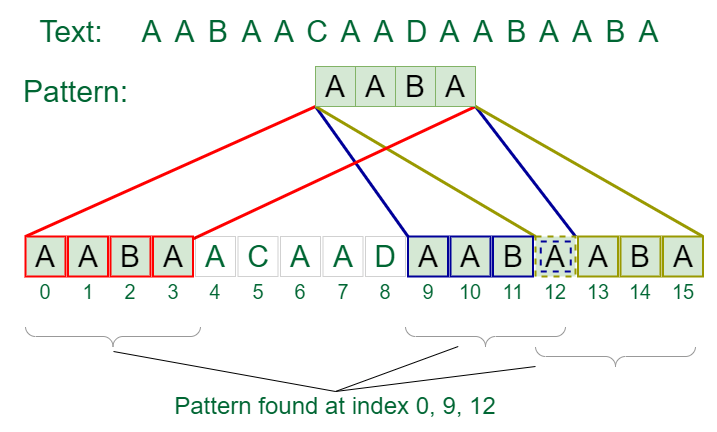Compare text characters with pattern characters

The time complexity of Naive Pattern Search method is O(m*n). The m is the size of pattern and n is the size of the main string.

## C++

 `// C++ program for Naive Pattern ` `// Searching algorithm ` `#include ` `using` `namespace` `std; ` ` `  `void` `search(``char``* pat, ``char``* txt) ` `{ ` `    ``int` `M = ``strlen``(pat); ` `    ``int` `N = ``strlen``(txt); ` ` `  `    ``/* A loop to slide pat[] one by one */` `    ``for` `(``int` `i = 0; i <= N - M; i++) { ` `        ``int` `j; ` ` `  `        ``/* For current index i, check for pattern match */` `        ``for` `(j = 0; j < M; j++) ` `            ``if` `(txt[i + j] != pat[j]) ` `                ``break``; ` ` `  `        ``if` `(j ` `            ``== M) ``// if pat[0...M-1] = txt[i, i+1, ...i+M-1] ` `            ``cout << ``"Pattern found at index "` `<< i << endl; ` `    ``} ` `} ` ` `  `// Driver's Code ` `int` `main() ` `{ ` `    ``char` `txt[] = ``"AABAACAADAABAAABAA"``; ` `    ``char` `pat[] = ``"AABA"``; ` ` `  `    ``// Function call ` `    ``search(pat, txt); ` `    ``return` `0; ` `}`

## Java

 `// Java program for Naive Pattern ` `// Searching algorithm ` `class` `GFG { ` ` `  `  ``static` `void` `search(``char``[] pat, ``char``[] txt) ` `  ``{ ` `    ``int` `M = pat.length; ` `    ``int` `N = txt.length; ` ` `  `    ``/* A loop to slide pat[] one by one */` `    ``for` `(``int` `i = ``0``; i <= N - M; i++) { ` `      ``int` `j; ` ` `  `      ``/* For current index i, check for pattern match ` `             ``*/` `      ``for` `(j = ``0``; j < M; j++) ` `        ``if` `(txt[i + j] != pat[j]) ` `          ``break``; ` ` `  `      ``// if pat[0...M-1] = txt[i, i+1, ...i+M-1] ` `      ``if` `(j == M) ` `        ``System.out.println(``"Pattern found at index "` `                           ``+ i); ` `    ``} ` `  ``} ` ` `  `  ``// Driver's Code ` ` `  `  ``public` `static` `void` `main(String[] args) ` `  ``{ ` `    ``char` `txt[] = ``"AABAACAADAABAAABAA"``.toCharArray(); ` ` `  `    ``char` `pat[] = ``"AABA"``.toCharArray(); ` ` `  `    ``// Function call ` `    ``search(pat, txt); ` `  ``} ` `} ` ` `  `// This code is contributed by karandeep1234`

## Python3

 `# Python program for above approach ` `def` `search(pat, txt): ` `    ``M ``=` `len``(pat) ` `    ``N ``=` `len``(txt) ` `    ``for` `i ``in` `range``(N``-``M): ` `        ``for` `j ``in` `range``(M): ` `            ``k ``=` `j``+``1` `            ``if``(txt[i``+``j] !``=` `pat[j]): ` `                ``break` `        ``if``(k ``=``=` `M): ` `            ``print``(``"Pattern found at index "``, i) ` ` `  `txt ``=` `"AABAACAADAABAAABAA"` `pat ``=` `"AABA"` `search(pat, txt) ` ` `  `# This code is contributed by ishankhandelwals.`

## C#

 `using` `System; ` ` `  `public` `class` `GFG { ` ` `  `  ``public` `static` `void` `search(``char``[] pat, ``char``[] txt) ` `  ``{ ` `    ``int` `M = pat.Length; ` `    ``int` `N = txt.Length; ` ` `  `    ``/* A loop to slide pat[] one by one */` `    ``for` `(``int` `i = 0; i <= N - M; i++) { ` `      ``int` `j; ` ` `  `      ``/* For current index i, check for pattern match ` `             ``*/` `      ``for` `(j = 0; j < M; j++) ` `        ``if` `(txt[i + j] != pat[j]) ` `          ``break``; ` ` `  `      ``if` `(j == M) ``// if pat[0...M-1] = txt[i, i+1, ` `        ``// ...i+M-1] ` `        ``Console.WriteLine(``"Pattern found at index "` `                          ``+ i); ` `    ``} ` `  ``} ` ` `  `  ``static` `public` `void` `Main() ` `  ``{ ` ` `  `    ``char``[] txt = ``"AABAACAADAABAAABAA"``.ToCharArray(); ` `    ``char``[] pat = ``"AABA"``.ToCharArray(); ` ` `  `    ``// Function call ` `    ``search(pat, txt); ` `  ``} ` `} ` `// This code is contributed by akashish__`

## Javascript

 `// JS program for Naive Pattern ` `// Searching algorithm ` `function` `search(pat, txt) ` `{ ` `    ``let M = pat.length; ` `    ``let N = txt.length; ` `     `  `    ``/* A loop to slide pat[] one by one */` `    ``for` `(let i = 0; i <= N - M; i++) { ` `        ``let j = 0; ` `         `  `        ``/* For current index i, check for pattern match */` `        ``for` `(j = 0; j < M; j++) ` `            ``if` `(txt[i + j] != pat[j]) ` `                ``break``; ` `        ``if` `(j == M) ``// if pat[0...M-1] = txt[i, i+1, ...i+M-1] ` `            ``console.log(``"Pattern found at index"``,i); ` `    ``} ` `} ` ` `  `// Driver's Code ` `    ``let txt = ``"AABAACAADAABAAABAA"``; ` `    ``let pat = ``"AABA"``; ` `     `  `    ``// Function call ` `    ``search(pat, txt); ` `     `  `    ``// This code is contributed by ishankhandelwals.`

Output

```Pattern found at index 0
Pattern found at index 9
Pattern found at index 13```

Time Complexity: O(N*M)
Auxiliary Space: O(1)

## KMP algorithm

KMP algorithm is used to find a “Pattern” in a “Text”. This algorithm compares character by character from left to right. But whenever a mismatch occurs, it uses a preprocessed table called “Prefix Table” to skip characters comparison while matching. Sometimes prefix table is also known as LPS Table. Here LPS stands for “Longest proper Prefix which is also Suffix”.

### How to use LPS Table

We use the LPS table to decide how many characters are to be skipped for comparison when a mismatch has occurred.
When a mismatch occurs, check the LPS value of the previous character of the mismatched character in the pattern. If it is ‘0’ then start comparing the first character of the pattern with the next character to the mismatched character in the text. If it is not ‘0’ then start comparing the character which is at an index value equal to the LPS value of the previous character to the mismatched character in pattern with the mismatched character in the Text.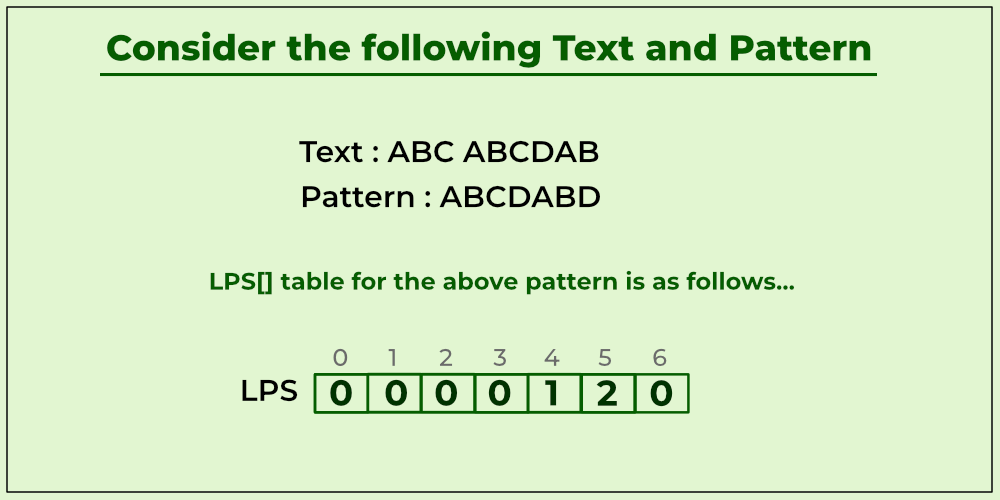Example of KMP algorithm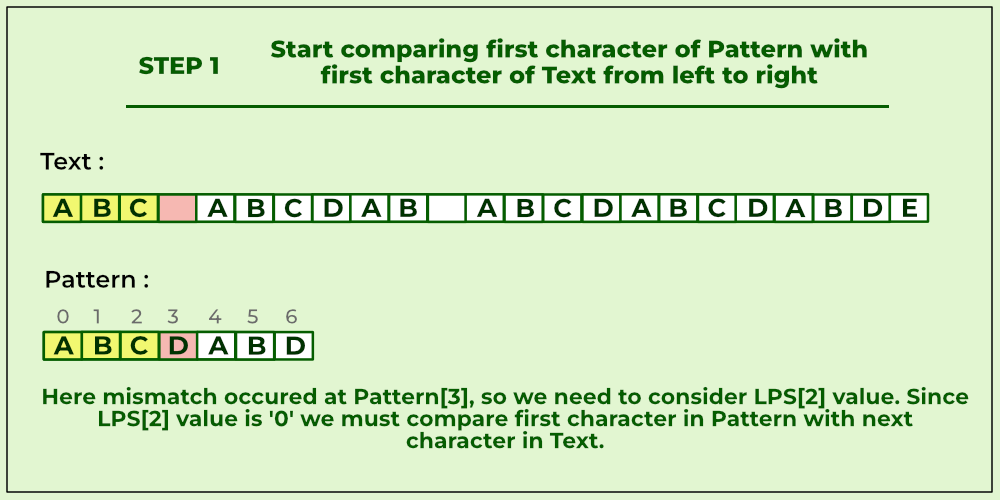Compare first character of pattern with first character of text from left to right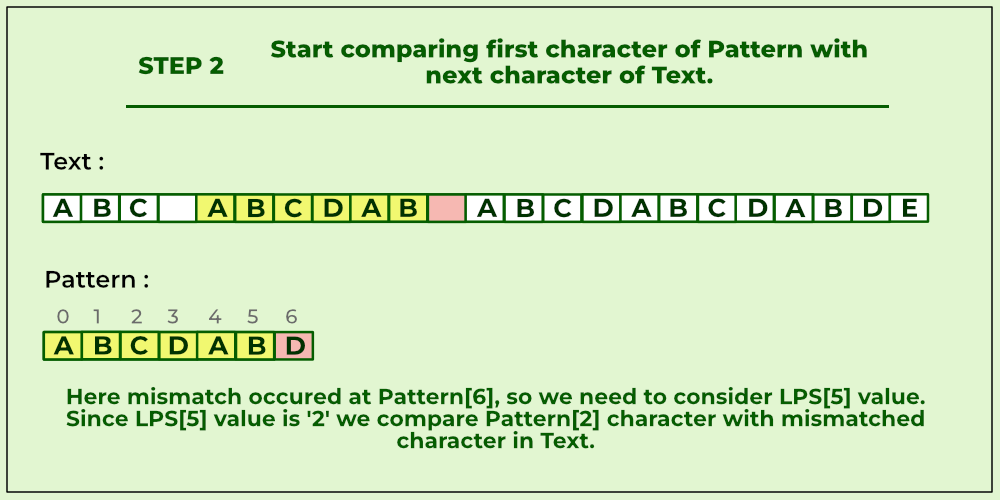Compare first character of pattern with next character of text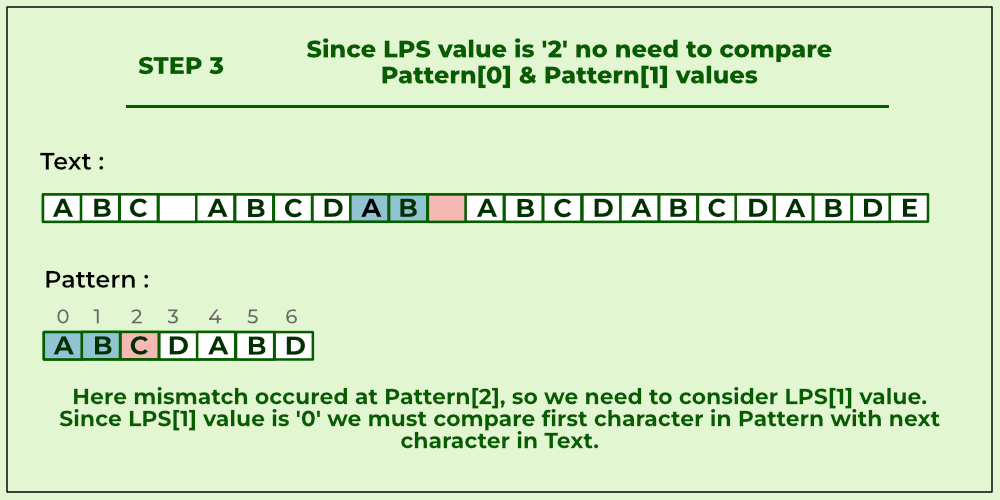Compare pattern and pattern values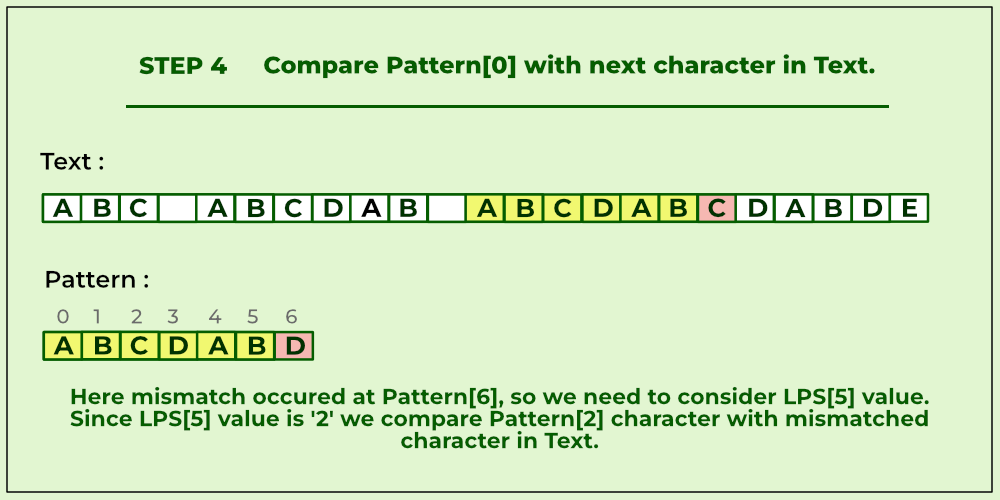Compare pattern with next characters in text.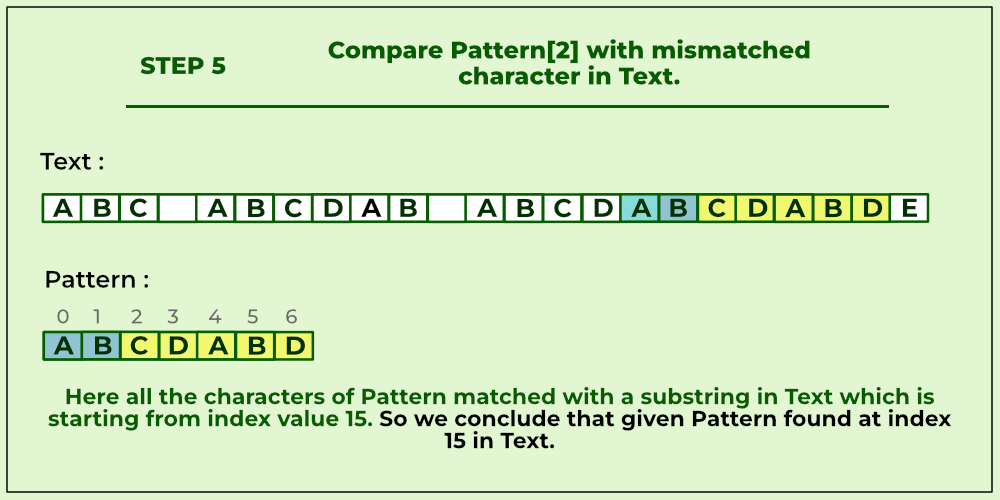Compare pattern with mismatched characters in text.

### How the KMP Algorithm Works

Let’s take a look on working example of KMP Algorithm to find a Pattern in a Text.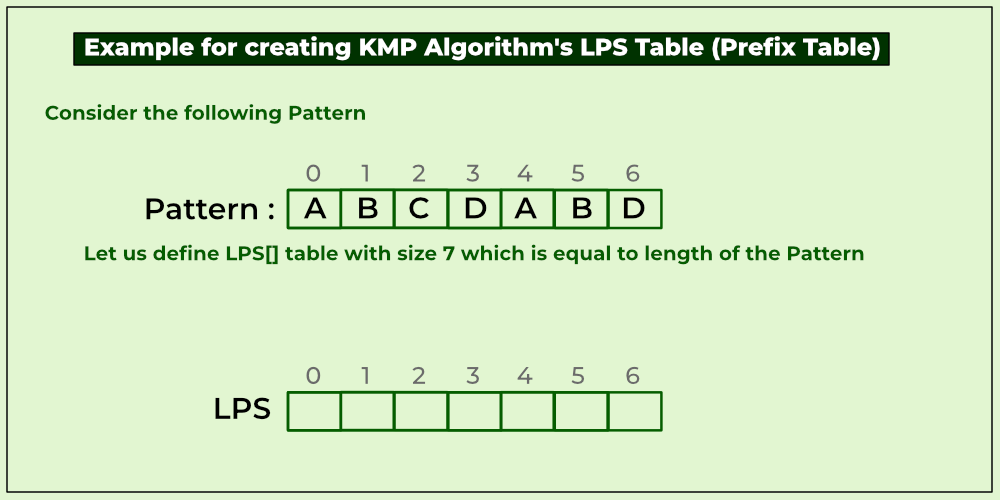LPS table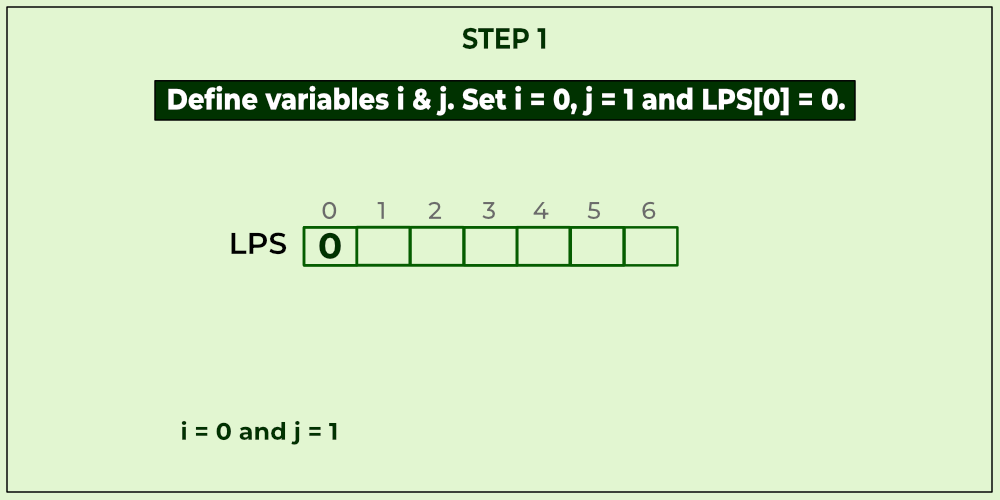Define variables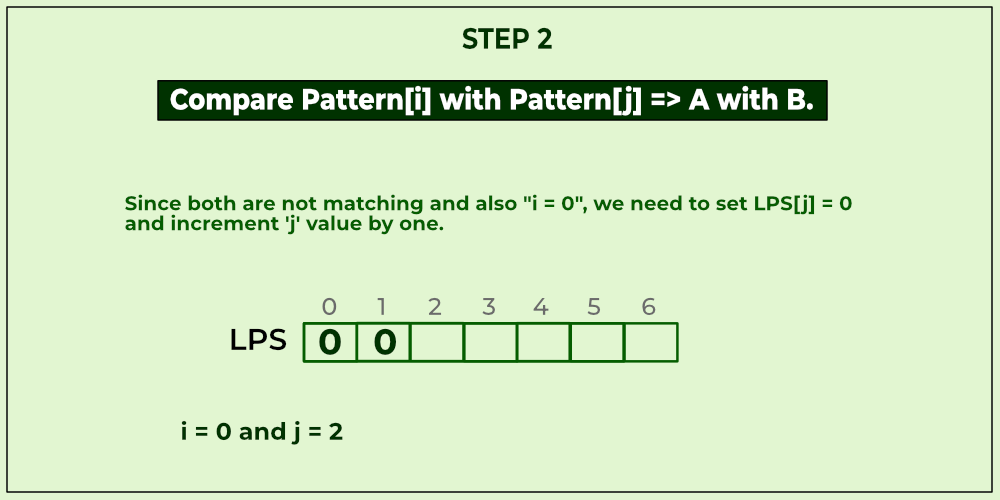Compare A with B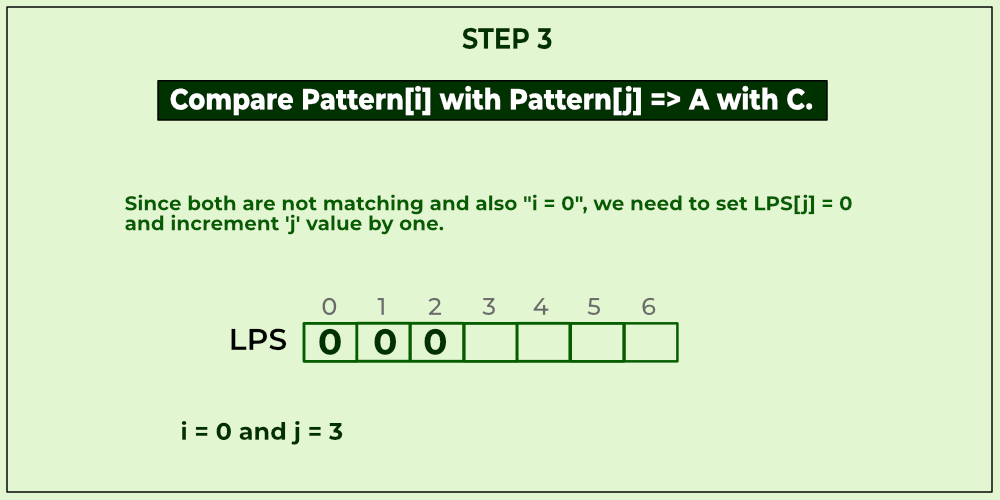Compare A with C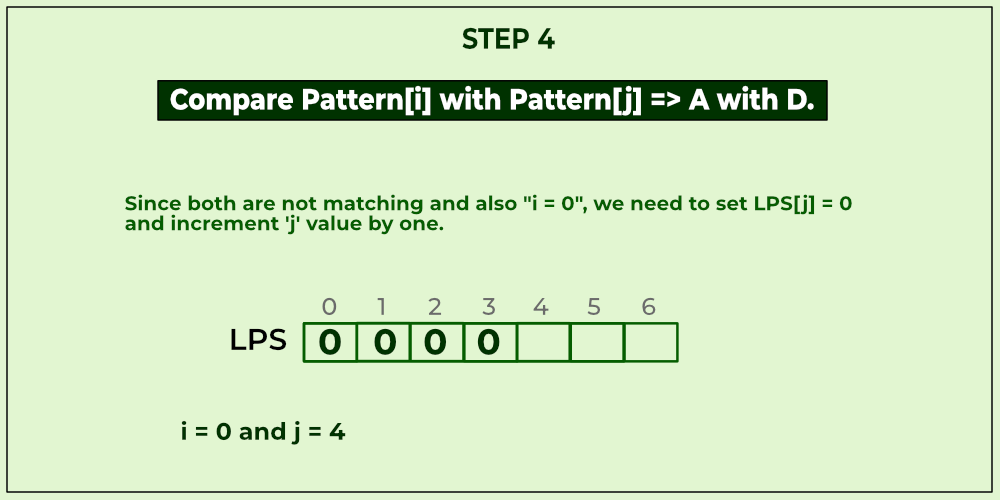Compare A with D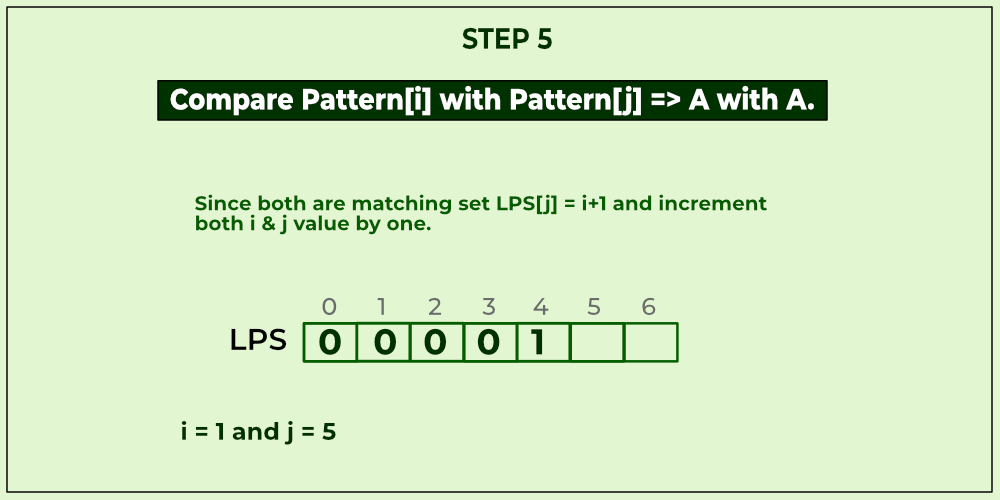Compare A with A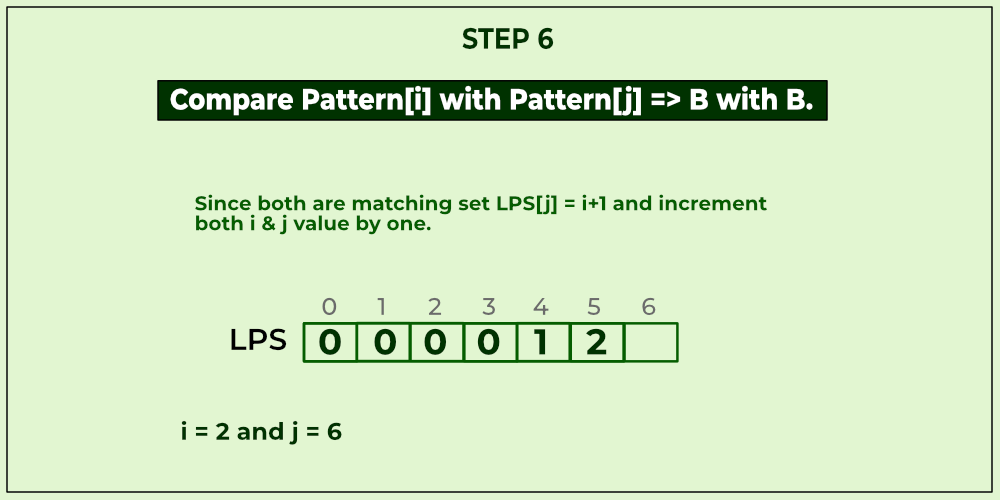Compare B with B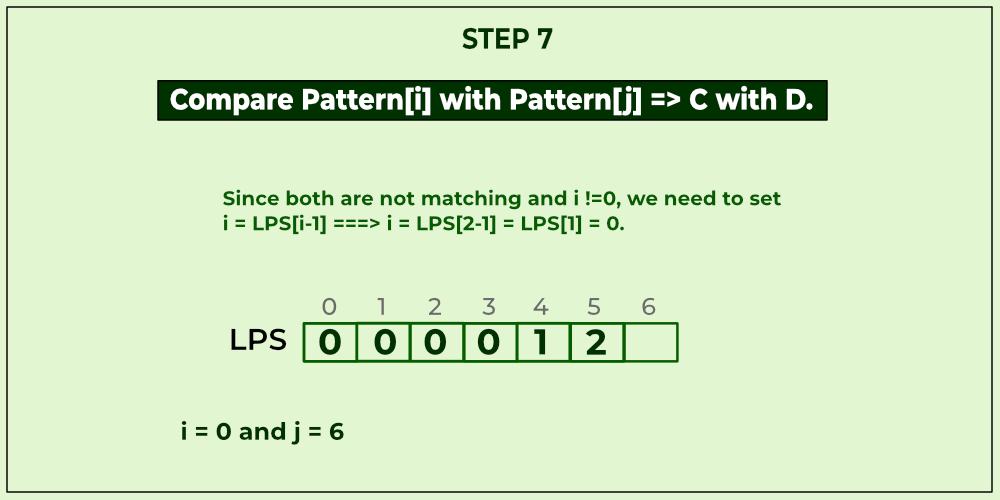Compare C with D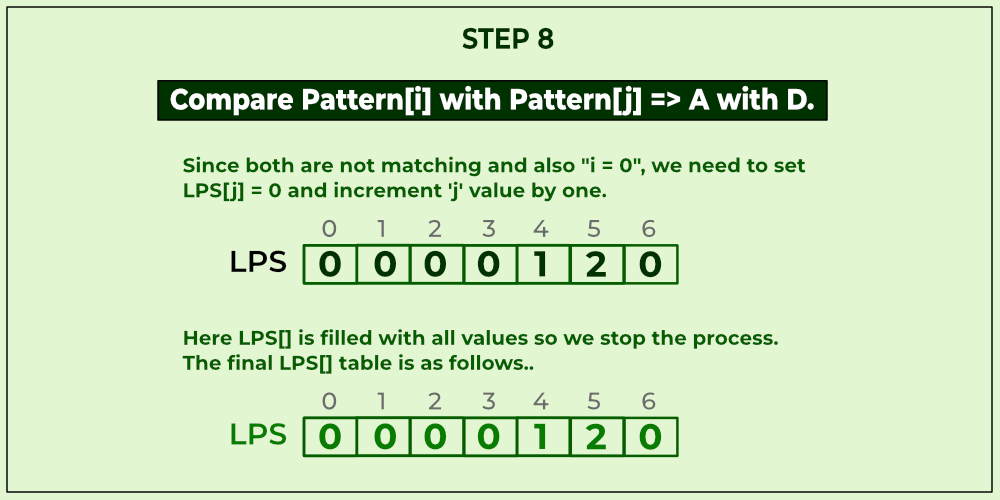Compare A with D

Implementation of the KMP algorithm:

## C++

 `// C++ program for implementation of KMP pattern searching ` `// algorithm ` `#include ` ` `  `void` `computeLPSArray(``char``* pat, ``int` `M, ``int``* lps); ` ` `  `// Prints occurrences of txt[] in pat[] ` `void` `KMPSearch(``char``* pat, ``char``* txt) ` `{ ` `    ``int` `M = ``strlen``(pat); ` `    ``int` `N = ``strlen``(txt); ` ` `  `    ``// create lps[] that will hold the longest prefix suffix ` `    ``// values for pattern ` `    ``int` `lps[M]; ` ` `  `    ``// Preprocess the pattern (calculate lps[] array) ` `    ``computeLPSArray(pat, M, lps); ` ` `  `    ``int` `i = 0; ``// index for txt[] ` `    ``int` `j = 0; ``// index for pat[] ` `    ``while` `((N - i) >= (M - j)) { ` `        ``if` `(pat[j] == txt[i]) { ` `            ``j++; ` `            ``i++; ` `        ``} ` ` `  `        ``if` `(j == M) { ` `            ``printf``(``"Found pattern at index %d "``, i - j); ` `            ``j = lps[j - 1]; ` `        ``} ` ` `  `        ``// mismatch after j matches ` `        ``else` `if` `(i < N && pat[j] != txt[i]) { ` `            ``// Do not match lps[0..lps[j-1]] characters, ` `            ``// they will match anyway ` `            ``if` `(j != 0) ` `                ``j = lps[j - 1]; ` `            ``else` `                ``i = i + 1; ` `        ``} ` `    ``} ` `} ` ` `  `// Fills lps[] for given pattern pat[0..M-1] ` `void` `computeLPSArray(``char``* pat, ``int` `M, ``int``* lps) ` `{ ` `    ``// length of the previous longest prefix suffix ` `    ``int` `len = 0; ` ` `  `    ``lps = 0; ``// lps is always 0 ` ` `  `    ``// the loop calculates lps[i] for i = 1 to M-1 ` `    ``int` `i = 1; ` `    ``while` `(i < M) { ` `        ``if` `(pat[i] == pat[len]) { ` `            ``len++; ` `            ``lps[i] = len; ` `            ``i++; ` `        ``} ` `        ``else` `// (pat[i] != pat[len]) ` `        ``{ ` `            ``// This is tricky. Consider the example. ` `            ``// AAACAAAA and i = 7. The idea is similar ` `            ``// to search step. ` `            ``if` `(len != 0) { ` `                ``len = lps[len - 1]; ` ` `  `                ``// Also, note that we do not increment ` `                ``// i here ` `            ``} ` `            ``else` `// if (len == 0) ` `            ``{ ` `                ``lps[i] = 0; ` `                ``i++; ` `            ``} ` `        ``} ` `    ``} ` `} ` ` `  `// Driver program to test above function ` `int` `main() ` `{ ` `    ``char` `txt[] = ``"ABABDABACDABABCABAB"``; ` `    ``char` `pat[] = ``"ABABCABAB"``; ` `    ``KMPSearch(pat, txt); ` `    ``return` `0; ` `} `

## Java

 `// Java program for implementation of KMP pattern searching  ` `// algorithm ` `public` `class` `KMP_String_Matching {  ` `    ``void` `KMPSearch(String pat, String txt)  ` `    ``{  ` `        ``int` `M = pat.length();  ` `        ``int` `N = txt.length();  ` ` `  `        ``// create lps[] that will hold the longest prefix suffix  ` `        ``// values for pattern  ` `        ``int` `lps[] = ``new` `int``[M];  ` `        ``int` `j = ``0``; ``// index for pat[]  ` ` `  `        ``// Preprocess the pattern (calculate lps[] array)  ` `        ``computeLPSArray(pat, M, lps);  ` ` `  `        ``int` `i = ``0``; ``// index for txt[]  ` `        ``while` `(i < N) {  ` `            ``if` `(pat.charAt(j) == txt.charAt(i)) {  ` `                ``j++;  ` `                ``i++;  ` `            ``}  ` `            ``if` `(j == M) {  ` `                ``System.out.println(``"Found pattern "` `+ ``"at index "` `+ (i - j));  ` `                ``j = lps[j - ``1``];  ` `            ``}  ` ` `  `            ``// mismatch after j matches  ` `            ``else` `if` `(i < N && pat.charAt(j) != txt.charAt(i)) {  ` `                ``// Do not match lps[0..lps[j-1]] characters,  ` `                ``// they will match anyway  ` `                ``if` `(j != ``0``)  ` `                    ``j = lps[j - ``1``];  ` `                ``else` `                    ``i = i + ``1``;  ` `            ``}  ` `        ``}  ` `    ``}  ` ` `  `    ``void` `computeLPSArray(String pat, ``int` `M, ``int` `lps[])  ` `    ``{  ` `        ``// length of the previous longest prefix suffix  ` `        ``int` `len = ``0``;  ` `        ``int` `i = ``1``;  ` `        ``lps[``0``] = ``0``; ``// lps is always 0  ` ` `  `        ``// the loop calculates lps[i] for i = 1 to M-1  ` `        ``while` `(i < M) {  ` `            ``if` `(pat.charAt(i) == pat.charAt(len)) {  ` `                ``len++;  ` `                ``lps[i] = len;  ` `                ``i++;  ` `            ``}  ` `            ``else` `// (pat[i] != pat[len])  ` `            ``{  ` `                ``// This is tricky. Consider the example.  ` `                ``// AAACAAAA and i = 7. The idea is similar  ` `                ``// to search step.  ` `                ``if` `(len != ``0``) {  ` `                    ``len = lps[len - ``1``];  ` ` `  `                    ``// Also, note that we do not increment  ` `                    ``// i here  ` `                ``}  ` `                ``else` `// if (len == 0)  ` `                ``{  ` `                    ``lps[i] = len;  ` `                    ``i++;  ` `                ``}  ` `            ``}  ` `        ``}  ` `    ``}  ` ` `  `    ``// Driver program to test above function  ` `    ``public` `static` `void` `main(String[] args)  ` `    ``{  ` `        ``String txt = ``"ABABDABACDABABCABAB"``;  ` `        ``String pat = ``"ABABCABAB"``;  ` `        ``new` `KMP_String_Matching().KMPSearch(pat, txt);  ` `    ``}  ` `}`

## Python3

 `# Python program for implementation of KMP pattern searching ` `# algorithm ` `def` `computeLPSArray(pat, M, lps): ` `    ``len` `=` `0`  `# length of the previous longest prefix suffix ` ` `  `    ``lps[``0``]  ``# lps is always 0 ` `    ``i ``=` `1` ` `  `    ``# the loop calculates lps[i] for i = 1 to M-1 ` `    ``while` `i < M: ` `        ``if` `pat[i] ``=``=` `pat[``len``]: ` `            ``len` `+``=` `1` `            ``lps[i] ``=` `len` `            ``i ``+``=` `1` `        ``else``: ` `            ``# This is tricky. Consider the example. ` `            ``# AAACAAAA and i = 7. The idea is similar ` `            ``# to search step. ` `            ``if` `len` `!``=` `0``: ` `                ``len` `=` `lps[``len``-``1``] ` ` `  `            ``# Also, note that we do not increment i here ` `            ``else``: ` `                ``lps[i] ``=` `0` `                ``i ``+``=` `1` ` `  `def` `KMPSearch(pat, txt): ` `    ``M ``=` `len``(pat) ` `    ``N ``=` `len``(txt) ` ` `  `    ``# create lps[] that will hold the longest prefix suffix ` `    ``# values for pattern ` `    ``lps ``=` `[``0``]``*``M ` `    ``j ``=` `0`  `# index for pat[] ` ` `  `    ``# Preprocess the pattern (calculate lps[] array) ` `    ``computeLPSArray(pat, M, lps) ` ` `  `    ``i ``=` `0`  `# index for txt[] ` `    ``while` `(N ``-` `i) >``=` `(M ``-` `j): ` `        ``if` `pat[j] ``=``=` `txt[i]: ` `            ``j ``+``=` `1` `            ``i ``+``=` `1` ` `  `        ``if` `j ``=``=` `M: ` `            ``print``(``"Found pattern at index:"``, i``-``j) ` `            ``j ``=` `lps[j``-``1``] ` ` `  `        ``# mismatch after j matches ` `        ``elif` `i < N ``and` `pat[j] !``=` `txt[i]: ` `            ``# Do not match lps[0..lps[j-1]] characters, ` `            ``# they will match anyway ` `            ``if` `j !``=` `0``: ` `                ``j ``=` `lps[j``-``1``] ` `            ``else``: ` `                ``i ``+``=` `1` ` `  `txt ``=` `"ABABDABACDABABCABAB"` `pat ``=` `"ABABCABAB"` `KMPSearch(pat, txt) ` ` `  `# This code is contributed by ishankhandelwals.`

## C#

 `using` `System; ` `using` `System.Collections.Generic; ` ` `  `public` `class` `GFG { ` ` `  `  ``// Prints occurrences of txt[] in pat[] ` `  ``public` `static` `void` `KMPSearch(``char``[] pat, ``char``[] txt) ` `  ``{ ` `    ``int` `M = pat.Length; ` `    ``int` `N = txt.Length; ` ` `  `    ``// create lps[] that will hold the longest prefix ` `    ``// suffix values for pattern ` `    ``int``[] lps = ``new` `int``[M]; ` ` `  `    ``// Preprocess the pattern (calculate lps[] array) ` `    ``computeLPSArray(pat, M, lps); ` ` `  `    ``int` `i = 0; ``// index for txt[] ` `    ``int` `j = 0; ``// index for pat[] ` `    ``while` `((N - i) >= (M - j)) { ` `      ``if` `(pat[j] == txt[i]) { ` `        ``j++; ` `        ``i++; ` `      ``} ` ` `  `      ``if` `(j == M) { ` `        ``int` `temp = i - j; ` `        ``Console.WriteLine(``"Found pattern at index "` `                          ``+ temp); ` `        ``j = lps[j - 1]; ` `      ``} ` ` `  `      ``// mismatch after j matches ` `      ``else` `if` `(i < N && pat[j] != txt[i]) { ` `        ``// Do not match lps[0..lps[j-1]] characters, ` `        ``// they will match anyway ` `        ``if` `(j != 0) ` `          ``j = lps[j - 1]; ` `        ``else` `          ``i = i + 1; ` `      ``} ` `    ``} ` `  ``} ` ` `  `  ``// Fills lps[] for given pattern pat[0..M-1] ` `  ``public` `static` `void` `computeLPSArray(``char``[] pat, ``int` `M, ` `                                     ``int``[] lps) ` `  ``{ ` `    ``// length of the previous longest prefix suffix ` `    ``int` `len = 0; ` ` `  `    ``lps = 0; ``// lps is always 0 ` ` `  `    ``// the loop calculates lps[i] for i = 1 to M-1 ` `    ``int` `i = 1; ` `    ``while` `(i < M) { ` `      ``if` `(pat[i] == pat[len]) { ` `        ``len++; ` `        ``lps[i] = len; ` `        ``i++; ` `      ``} ` `      ``else` `// (pat[i] != pat[len]) ` `      ``{ ` `        ``// This is tricky. Consider the example. ` `        ``// AAACAAAA and i = 7. The idea is similar ` `        ``// to search step. ` `        ``if` `(len != 0) { ` `          ``len = lps[len - 1]; ` ` `  `          ``// Also, note that we do not increment ` `          ``// i here ` `        ``} ` `        ``else` `// if (len == 0) ` `        ``{ ` `          ``lps[i] = 0; ` `          ``i++; ` `        ``} ` `      ``} ` `    ``} ` `  ``} ` ` `  `  ``static` `public` `void` `Main() ` `  ``{ ` ` `  `    ``char``[] txt = ``"ABABDABACDABABCABAB"``.ToCharArray(); ` `    ``char``[] pat = ``"ABABCABAB"``.ToCharArray(); ` `    ``KMPSearch(pat, txt); ` `  ``} ` `} ` ` `  `// This code is contributed by akashish__`

## Javascript

 `// JS program for implementation of KMP pattern searching ` `// algorithm ` `// Prlets occurrences of txt[] in pat[] ` `function` `computeLPSArray(pat, M, lps) ` `{ ` ` `  `    ``// length of the previous longest prefix suffix ` `    ``let len = 0; ` `    ``lps = 0; ``// lps is always 0 ` `    ``// the loop calculates lps[i] for i = 1 to M-1 ` `    ``let i = 1; ` `    ``while` `(i < M) { ` `        ``if` `(pat[i] == pat[len]) { ` `            ``len++; ` `            ``lps[i] = len; ` `            ``i++; ` `        ``} ` `        ``else` `// (pat[i] != pat[len]) ` `        ``{ ` `         `  `            ``// This is tricky. Consider the example. ` `            ``// AAACAAAA and i = 7. The idea is similar ` `            ``// to search step. ` `            ``if` `(len != 0) { ` `                ``len = lps[len - 1]; ` `                 `  `                ``// Also, note that we do not increment ` `                ``// i here ` `            ``} ` `            ``else` `// if (len == 0) ` `            ``{ ` `                ``lps[i] = 0; ` `                ``i++; ` `            ``} ` `        ``} ` `    ``} ` `} ` `function` `KMPSearch(pat, txt) { ` `    ``let M = pat.length; ` `    ``let N = txt.length ` `     `  `    ``// create lps[] that will hold the longest prefix suffix ` `    ``// values for pattern ` `    ``let lps = []; ` `     `  `    ``// Preprocess the pattern (calculate lps[] array) ` `    ``computeLPSArray(pat, M, lps); ` `    ``let i = 0; ``// index for txt[] ` `    ``let j = 0; ``// index for pat[] ` `    ``while` `((N - i) >= (M - j)) { ` `        ``if` `(pat[j] == txt[i]) { ` `            ``j++; ` `            ``i++; ` `        ``} ` `        ``if` `(j == M) { ` `            ``console.log(``"Found pattern at index:"``, i - j); ` `            ``j = lps[j - 1]; ` `        ``} ` `         `  `        ``// mismatch after j matches ` `        ``else` `if` `(i < N && pat[j] != txt[i]) ` `        ``{ ` `         `  `            ``// Do not match lps[0..lps[j-1]] characters, ` `            ``// they will match anyway ` `            ``if` `(j != 0) ` `                ``j = lps[j - 1]; ` `            ``else` `                ``i = i + 1; ` `        ``} ` `    ``} ` `} ` ` `  `// Fills lps[] for given pattern pat[0..M-1] ` `// Driver program to test above function ` `let txt = ``"ABABDABACDABABCABAB"``; ` `let pat = ``"ABABCABAB"``; ` `KMPSearch(pat, txt); ` ` `  `// This code is contributed by ishankhandelwals.`

Output

`Found pattern at index 10 `

Time complexity: O(n + m)
Auxiliary Space: O(M)

## Rabin Karp algorithm:

Rabin-Karp algorithm is an algorithm used for searching/matching patterns in the text using a hash function. Unlike Naive string-matching algorithm, it does not travel through every character in the initial phase rather it filters the characters that do not match and then perform the comparison.

Rabin-Karp compares a string’s hash values, rather than the strings themselves. For efficiency, the hash value of the next position in the text is easily computed from the hash value of the current position.

### Working of Rabin-Karp algorithm

• Initially calculate the hash value of the pattern P.
• Start iterating from the start of the string:
• Calculate the hash value of the current substring having length m.
• If the hash value of the current substring and the pattern are same check if the substring is same as the pattern.
• If they are same, store the starting index as a valid answer. Otherwise, continue for the next substrings.
• Return the starting indices as the required answer.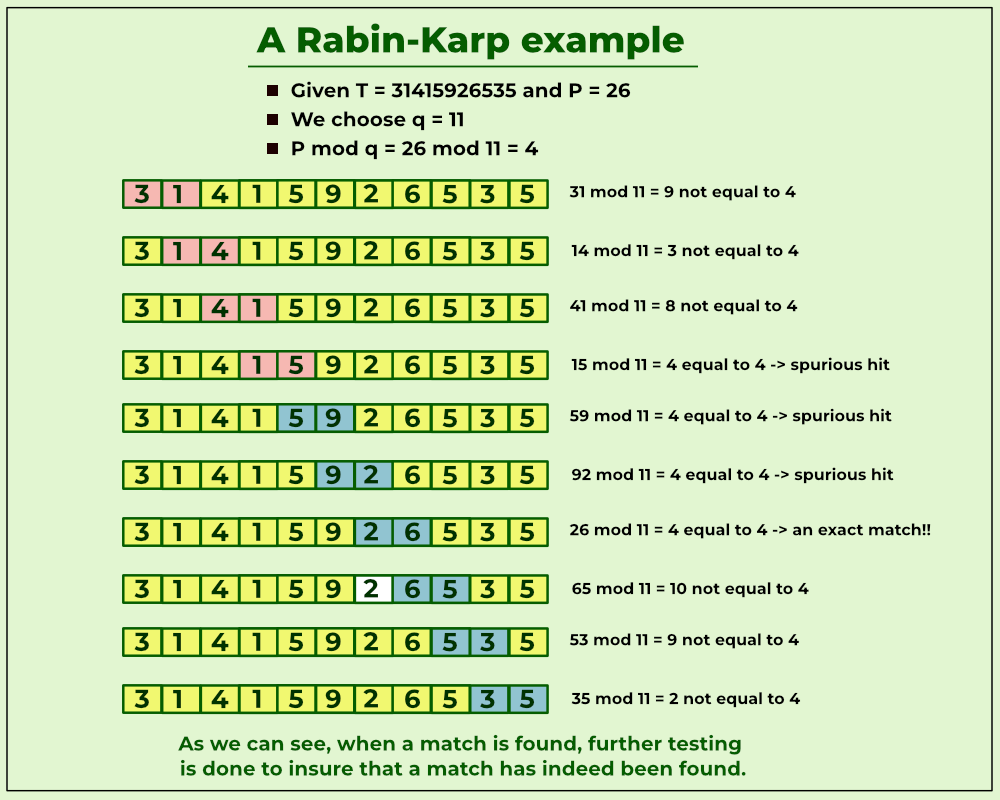Example of Rabin Karp

Below is the implementation of the Rabin-Karp algorithm.

## C++

 `/* Following program is a C++ implementation of Rabin Karp ` `Algorithm given in the CLRS book */` `#include ` `using` `namespace` `std; ` ` `  `// d is the number of characters in the input alphabet ` `#define d 256 ` ` `  `/* pat -> pattern ` `    ``txt -> text ` `    ``q -> A prime number ` `*/` `void` `search(``char` `pat[], ``char` `txt[], ``int` `q) ` `{ ` `    ``int` `M = ``strlen``(pat); ` `    ``int` `N = ``strlen``(txt); ` `    ``int` `i, j; ` `    ``int` `p = 0; ``// hash value for pattern ` `    ``int` `t = 0; ``// hash value for txt ` `    ``int` `h = 1; ` ` `  `    ``// The value of h would be "pow(d, M-1)%q" ` `    ``for` `(i = 0; i < M - 1; i++) ` `        ``h = (h * d) % q; ` ` `  `    ``// Calculate the hash value of pattern and first ` `    ``// window of text ` `    ``for` `(i = 0; i < M; i++) { ` `        ``p = (d * p + pat[i]) % q; ` `        ``t = (d * t + txt[i]) % q; ` `    ``} ` ` `  `    ``// Slide the pattern over text one by one ` `    ``for` `(i = 0; i <= N - M; i++) { ` ` `  `        ``// Check the hash values of current window of text ` `        ``// and pattern. If the hash values match then only ` `        ``// check for characters one by one ` `        ``if` `(p == t) { ` `            ``/* Check for characters one by one */` `            ``for` `(j = 0; j < M; j++) { ` `                ``if` `(txt[i + j] != pat[j]) { ` `                    ``break``; ` `                ``} ` `            ``} ` ` `  `            ``// if p == t and pat[0...M-1] = txt[i, i+1, ` `            ``// ...i+M-1] ` ` `  `            ``if` `(j == M) ` `                ``cout << ``"Pattern found at index "` `<< i ` `                     ``<< endl; ` `        ``} ` ` `  `        ``// Calculate hash value for next window of text: ` `        ``// Remove leading digit, add trailing digit ` `        ``if` `(i < N - M) { ` `            ``t = (d * (t - txt[i] * h) + txt[i + M]) % q; ` ` `  `            ``// We might get negative value of t, converting ` `            ``// it to positive ` `            ``if` `(t < 0) ` `                ``t = (t + q); ` `        ``} ` `    ``} ` `} ` ` `  `/* Driver code */` `int` `main() ` `{ ` `    ``char` `txt[] = ``"GEEKS FOR GEEKS"``; ` `    ``char` `pat[] = ``"GEEK"``; ` ` `  `    ``// we mod to avoid overflowing of value but we should ` `    ``// take as big q as possible to avoid the collison ` `    ``int` `q = INT_MAX; ` ` `  `    ``// Function Call ` `    ``search(pat, txt, q); ` `    ``return` `0; ` `} ` ` `  `// This is code is contributed by rathbhupendra `

## Java

 `import` `java.io.*; ` `import` `java.lang.*; ` `import` `java.util.*; ` ` `  `/* pat -> pattern ` `    ``txt -> text ` `    ``q -> A prime number ` `*/` `public` `class` `GFG { ` `  ``// d is the number of characters in the input alphabet ` `  ``public` `final` `static` `int` `d = ``256``; ` `  ``public` `static` `void` `search(String pat, String txt, ``int` `q) ` `  ``{ ` `    ``int` `M = pat.length(); ` `    ``int` `N = txt.length(); ` `    ``int` `i, j; ` `    ``int` `p = ``0``; ``// hash value for pattern ` `    ``int` `t = ``0``; ``// hash value for txt ` `    ``int` `h = ``1``; ` ` `  `    ``// The value of h would be "pow(d, M-1)%q" ` `    ``for` `(i = ``0``; i < M - ``1``; i++) ` `      ``h = (h * d) % q; ` `    ``// Calculate the hash value of pattern and first ` `    ``// window of text ` `    ``for` `(i = ``0``; i < M; i++) { ` `      ``p = (d * p + pat.charAt(i)) % q; ` `      ``t = (d * t + txt.charAt(i)) % q; ` `    ``} ` ` `  `    ``// Slide the pattern over text one by one ` `    ``for` `(i = ``0``; i <= N - M; i++) { ` ` `  `      ``// Check the hash values of current window of ` `      ``// text and pattern. If the hash values match ` `      ``// then only check for characters one by one ` `      ``if` `(p == t) { ` `        ``/* Check for characters one by one */` `        ``for` `(j = ``0``; j < M; j++) { ` `          ``if` `(txt.charAt(i + j) ` `              ``!= pat.charAt(j)) { ` `            ``break``; ` `          ``} ` `        ``} ` ` `  `        ``// if p == t and pat[0...M-1] = txt[i, i+1, ` `        ``// ...i+M-1] ` ` `  `        ``if` `(j == M) { ` `          ``System.out.println( ` `            ``"Pattern found at index "` `+ i); ` `        ``} ` `      ``} ` `      ``// Calculate hash value for next window of text: ` `      ``// Remove leading digit, add trailing digit ` `      ``if` `(i < N - M) { ` `        ``t = (d * (t - txt.charAt(i) * h) ` `             ``+ txt.charAt(i + M)) ` `          ``% q; ` ` `  `        ``// We might get negative value of t, ` `        ``// converting it to positive ` `        ``if` `(t < ``0``) ` `          ``t = (t + q); ` `      ``} ` `    ``} ` `  ``} ` ` `  `  ``/* Driver code */` `  ``public` `static` `void` `main(String[] args) ` `  ``{ ` `    ``String txt = ``"GEEKS FOR GEEKS"``; ` `    ``String pat = ``"GEEK"``; ` ` `  `    ``// A prime number ` `    ``int` `q = ``101``; ` ` `  `    ``// Function Call ` `    ``search(pat, txt, q); ` `  ``} ` `} ` ` `  `// This code is contributed by ishankhandelwals.`

## Python3

 `# d is the number of characters in the input alphabet ` `d ``=` `256` ` `  `''' pat -> pattern ` `txt -> text ` `q -> A prime number '''` `def` `search(pat, txt, q): ` `     `  `    ``M ``=` `len``(pat) ` `    ``N ``=` `len``(txt) ` `    ``p ``=` `0` `# hash value for pattern ` `    ``t ``=` `0` `# hash value for txt ` `    ``h ``=` `1` ` `  `    ``# The value of h would be "pow(d, M-1)%q" ` `    ``for` `i ``in` `range``(M ``-` `1``): ` `        ``h ``=` `(h ``*` `d) ``%` `q ` ` `  `    ``# Calculate the hash value of pattern and first ` `    ``# window of text ` `    ``for` `i ``in` `range``(M): ` `        ``p ``=` `(d ``*` `p ``+` `ord``(pat[i])) ``%` `q ` `        ``t ``=` `(d ``*` `t ``+` `ord``(txt[i])) ``%` `q ` ` `  `    ``# Slide the pattern over text one by one ` `    ``for` `i ``in` `range``(N ``-` `M ``+` `1``): ` `        ``# Check the hash values of current window of text ` `        ``# and pattern. If the hash values match then only ` `        ``# check for characters one by one ` `        ``if` `p ``=``=` `t: ` `            ``# Check for characters one by one ` `            ``for` `j ``in` `range``(M): ` `                ``if` `txt[i ``+` `j] !``=` `pat[j]: ` `                    ``break` `            ``# if p == t and pat[0...M-1] = txt[i, i+1, ` `            ``# ...i+M-1] ` `            ``if` `j ``=``=` `M ``-` `1``: ` `                ``print``(``"Pattern found at index "` `+` `str``(i)) ` ` `  `        ``# Calculate hash value for next window of text: ` `        ``# Remove leading digit, add trailing digit ` `        ``if` `i < N ``-` `M: ` `            ``t ``=` `(d ``*` `(t ``-` `ord``(txt[i]) ``*` `h) ``+` `ord``(txt[i ``+` `M])) ``%` `q ` `            ``# We might get negative value of t, converting ` `            ``# it to positive ` `            ``if` `t < ``0``: ` `                ``t ``=` `(t ``+` `q) ` ` `  `# Driver code ` `txt ``=` `"GEEKS FOR GEEKS"` `pat ``=` `"GEEK"` ` `  `# we mod to avoid overflowing of value but we should ` `# take as big q as possible to avoid the collison ` `q ``=` `float``(``'inf'``) ` ` `  `# Function Call ` `search(pat, txt, q) ` ` `  `# This code is contributed by akashish__ `

## C#

 `// C# code ` `using` `System; ` ` `  `/* pat -> pattern ` `    ``txt -> text ` `    ``q -> A prime number ` `*/` ` `  `class` `GFG { ` `  ``// d is the number of characters in the input alphabet ` `  ``public` `static` `int` `d = 256; ` `  ``public` `static` `void` `search(``string` `pat, ``string` `txt, ``int` `q) ` `  ``{ ` `    ``int` `M = pat.Length; ` `    ``int` `N = txt.Length; ` `    ``int` `i, j; ` `    ``int` `p = 0; ``// hash value for pattern ` `    ``int` `t = 0; ``// hash value for txt ` `    ``int` `h = 1; ` `    ``// The value of h would be "pow(d, M-1)%q" ` `    ``for` `(i = 0; i < M - 1; i++) ` `      ``h = (h * d) % q; ` `    ``// Calculate the hash value of pattern and first ` `    ``// window of text ` `    ``for` `(i = 0; i < M; i++) { ` `      ``p = (d * p + pat[i]) % q; ` `      ``t = (d * t + txt[i]) % q; ` `    ``} ` ` `  `    ``// Slide the pattern over text one by one ` `    ``for` `(i = 0; i <= N - M; i++) { ` ` `  `      ``// Check the hash values of current window of ` `      ``// text and pattern. If the hash values match ` `      ``// then only check for characters one by one ` `      ``if` `(p == t) { ` `        ``/* Check for characters one by one */` `        ``for` `(j = 0; j < M; j++) { ` `          ``if` `(txt[i + j] != pat[j]) { ` `            ``break``; ` `          ``} ` `        ``} ` ` `  `        ``// if p == t and pat[0...M-1] = txt[i, i+1, ` `        ``// ...i+M-1] ` ` `  `        ``if` `(j == M) { ` `          ``Console.WriteLine( ` `            ``"Pattern found at index "` `+ i); ` `        ``} ` `      ``} ` `      ``// Calculate hash value for next window of text: ` `      ``// Remove leading digit, add trailing digit ` `      ``if` `(i < N - M) { ` `        ``t = (d * (t - txt[i] * h) + txt[i + M]) % q; ` ` `  `        ``// We might get negative value of t, ` `        ``// converting it to positive ` `        ``if` `(t < 0) ` `          ``t = (t + q); ` `      ``} ` `    ``} ` `  ``} ` ` `  `  ``/* Driver code */` `  ``public` `static` `void` `Main(``string``[] args) ` `  ``{ ` `    ``string` `txt = ``"GEEKS FOR GEEKS"``; ` `    ``string` `pat = ``"GEEK"``; ` ` `  `    ``// A prime number ` `    ``int` `q = 101; ` ` `  `    ``// Function Call ` `    ``search(pat, txt, q); ` `  ``} ` `} ` ` `  `// This code is contributed by akashish__`

## Javascript

 `// d is the number of characters in the input alphabet ` `const d = 256; ` ` `  `/* pat -> pattern ` `    ``txt -> text ` `    ``q -> A prime number ` `*/` `function` `search(pat, txt, q) { ` `  ``const M = pat.length; ` `  ``const N = txt.length; ` `  ``let p = 0; ``// hash value for pattern ` `  ``let t = 0; ``// hash value for txt ` `  ``let h = 1; ` ` `  `  ``// The value of h would be "pow(d, M-1)%q" ` `  ``for` `(let i = 0; i < M - 1; i++) { ` `    ``h = (h * d) % q; ` `  ``} ` ` `  `  ``// Calculate the hash value of pattern and first ` `  ``// window of text ` `  ``for` `(let i = 0; i < M; i++) { ` `    ``p = (d * p + pat.charCodeAt(i)) % q; ` `    ``t = (d * t + txt.charCodeAt(i)) % q; ` `  ``} ` ` `  `  ``// Slide the pattern over text one by one ` `  ``for` `(let i = 0; i <= N - M; i++) { ` `    ``// Check the hash values of current window of text ` `    ``// and pattern. If the hash values match then only ` `    ``// check for characters one by one ` `    ``if` `(p === t) { ` `    ``/* Check for characters one by one */` `    ``for` `(j = 0; j < M; j++) { ` `        ``if` `(txt.charAt(i + j) !== pat.charAt(j)) { ` `        ``break``; ` `        ``} ` `    ``} ` ` `  `    ``// if p == t and pat[0...M-1] = txt[i, i+1, ` `    ``// ...i+M-1] ` ` `  `    ``if` `(j === M) ` `        ``console.log(``"Pattern found at index "` `+ i); ` `    ``} ` ` `  `    ``// Calculate hash value for next window of text: ` `    ``// Remove leading digit, add trailing digit ` `    ``if` `(i < N - M) { ` `    ``t = (d * (t - txt.charCodeAt(i) * h) + txt.charCodeAt(i + M)) % q; ` ` `  `    ``// We might get negative value of t, converting ` `    ``// it to positive ` `    ``if` `(t < 0) ` `        ``t = (t + q); ` `    ``} ` `  ``} ` `} ` ` `  `/* Driver code */` `const txt = ``"GEEKS FOR GEEKS"``; ` `const pat = ``"GEEK"``; ` ` `  `// we mod to avoid overflowing of value but we should ` `// take as big q as possible to avoid the collison ` `const q = Number.MAX_SAFE_INTEGER; ` ` `  `// Function Call ` `search(pat, txt, q); ` ` `  `// This code is contributed by ishankhandelwals.`

Output

```Pattern found at index 0
Pattern found at index 10```

Time Complexity:

• The average and best-case running time of the Rabin-Karp algorithm is O(n+m), but its worst-case time is O(nm).
• The worst case of the Rabin-Karp algorithm occurs when all characters of pattern and text are the same as the hash values of all the substrings of txt[] match with the hash value of pat[].

Space Complexity :

The space complexity of the Rabin-Karp algorithm is O(1), which means that it is a constant amount of memory that is required, regardless of the size of the input text and pattern. This is because the algorithm only needs to store a few variables that are updated as the algorithm progresses through the text and pattern. Specifically, the algorithm needs to store the hash value of the pattern, the hash value of the current window in the text, and a few loop counters and temporary variables. Since the size of these variables is fixed, the space complexity is constant.

## Z algorithm:

This algorithm finds all occurrences of a pattern in a text in linear time. Let length of text be n and of pattern be m, then total time taken is O(m + n) with linear space complexity. Z algorithm works by maintaining an auxiliary array called the Z array. This Z array stores the length of the longest substring, starting from the current index, that also it’s prefix.

### What is Z Array?

For a string str[0..n-1], Z array is of same length as string. An element Z[i] of Z array stores length of the longest substring starting from str[i] which is also a prefix of str[0..n-1]. The first entry of Z array is meaning less as complete string is always prefix of itself.

Example:

Index            0   1   2   3   4   5   6   7   8   9  10  11
Text             a   a   b   c   a   a   b   x   a   a   a   z
Z values         X   1   0   0   3   1   0   0   2   2   1   0

### How to construct Z array?

A Simple Solution is to run two nested loops, the outer loop goes to every index and the inner loop finds length of the longest prefix that matches the substring starting at current index. The time complexity of this solution is O(n2).

We can construct Z array in linear time. The idea is to maintain an interval [L, R] which is the interval with max R
such that [L, R] is prefix substring (substring which is also a prefix.

Steps for maintaining this interval are as follows –

1. If i > R then there is no prefix substring that starts before i and ends after i, so we reset L and R and compute new [L, R] by comparing str[0..] to str[i..] and get Z[i] (= R-L+1).
2. If i <= R then let K = i-L,  now Z[i] >= min(Z[K], R-i+1)  because str[i..] matches with str[K..] for atleast R-i+1 characters (they are in[L, R] interval which we know is a prefix substring).
Now two sub cases arise:
• If Z[K] < R-i+1  then there is no prefix substring starting at str[i] (otherwise Z[K] would be larger)  so  Z[i] = Z[K]and interval [L, R] remains same.
• If Z[K] >= R-i+1 then it is possible to extend the [L, R] interval thus we will set L as i and start matching from str[R] onwards  and get new R then we will update interval [L, R] and calculate Z[i] (=R-L+1).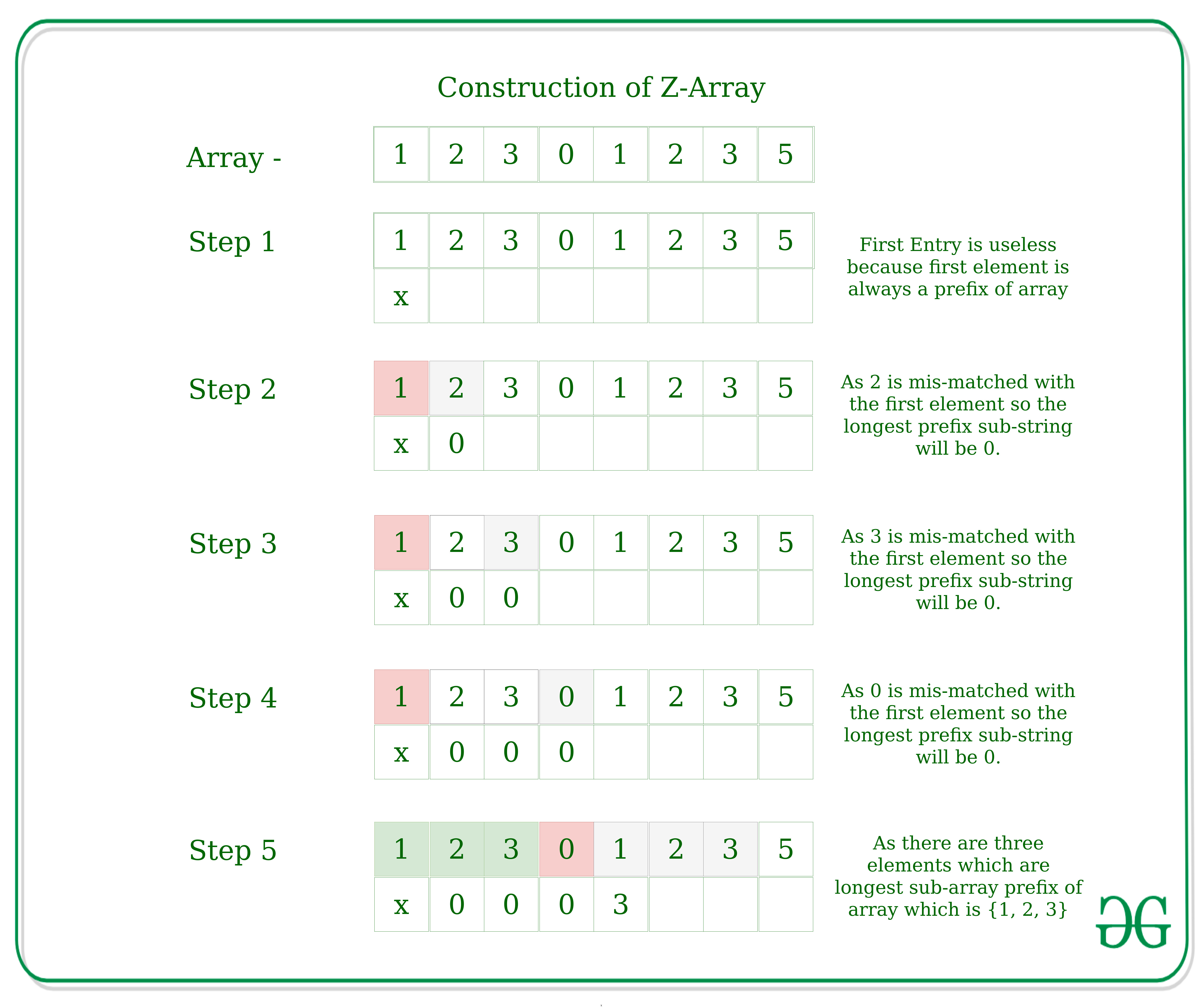Construction of Z array

Below is the implementation of the Z algorithm:

## C++

 `// A C++ program that implements Z algorithm for pattern ` `// searching ` `#include ` `using` `namespace` `std; ` ` `  `void` `getZarr(string str, ``int` `Z[]); ` ` `  `// prints all occurrences of pattern in text using Z algo ` `void` `search(string text, string pattern) ` `{ ` `    ``// Create concatenated string "P\$T" ` `    ``string concat = pattern + ``"\$"` `+ text; ` `    ``int` `l = concat.length(); ` ` `  `    ``// Construct Z array ` `    ``int` `Z[l]; ` `    ``getZarr(concat, Z); ` ` `  `    ``// now looping through Z array for matching condition ` `    ``for` `(``int` `i = 0; i < l; ++i) { ` `        ``// if Z[i] (matched region) is equal to pattern ` `        ``// length we got the pattern ` `        ``if` `(Z[i] == pattern.length()) ` `            ``cout << ``"Pattern found at index "` `                 ``<< i - pattern.length() - 1 << endl; ` `    ``} ` `} ` ` `  `// Fills Z array for given string str[] ` `void` `getZarr(string str, ``int` `Z[]) ` `{ ` `    ``int` `n = str.length(); ` `    ``int` `L, R, k; ` ` `  `    ``// [L, R] make a window which matches with prefix of s ` `    ``L = R = 0; ` `    ``for` `(``int` `i = 1; i < n; ++i) { ` `        ``// if i>R nothing matches so we will calculate. ` `        ``// Z[i] using naive way. ` `        ``if` `(i > R) { ` `            ``L = R = i; ` ` `  `            ``// R-L = 0 in starting, so it will start ` `            ``// checking from 0'th index. For example, ` `            ``// for "ababab" and i = 1, the value of R ` `            ``// remains 0 and Z[i] becomes 0. For string ` `            ``// "aaaaaa" and i = 1, Z[i] and R become 5 ` `            ``while` `(R < n && str[R - L] == str[R]) ` `                ``R++; ` `            ``Z[i] = R - L; ` `            ``R--; ` `        ``} ` `        ``else` `{ ` `            ``// k = i-L so k corresponds to number which ` `            ``// matches in [L, R] interval. ` `            ``k = i - L; ` ` `  `            ``// if Z[k] is less than remaining interval ` `            ``// then Z[i] will be equal to Z[k]. ` `            ``// For example, str = "ababab", i = 3, R = 5 ` `            ``// and L = 2 ` `            ``if` `(Z[k] < R - i + 1) ` `                ``Z[i] = Z[k]; ` ` `  `            ``// For example str = "aaaaaa" and i = 2, R is 5, ` `            ``// L is 0 ` `            ``else` `{ ` `                ``// else start from R and check manually ` `                ``L = i; ` `                ``while` `(R < n && str[R - L] == str[R]) ` `                    ``R++; ` `                ``Z[i] = R - L; ` `                ``R--; ` `            ``} ` `        ``} ` `    ``} ` `} ` ` `  `// Driver program ` `int` `main() ` `{ ` `    ``string text = ``"GEEKS FOR GEEKS"``; ` `    ``string pattern = ``"GEEK"``; ` `    ``search(text, pattern); ` `    ``return` `0; ` `}`

## Java

 `// A Java program that implements Z algorithm for pattern ` `// searching ` `import` `java.io.*; ` ` `  `class` `GFG  ` `{ ` ` `  `  ``// prints all occurrences of pattern in text using Z ` `  ``// algo ` `  ``static` `void` `search(String text, String pattern) ` `  ``{ ` ` `  `    ``// Create concatenated string "P\$T" ` `    ``String concat = pattern + ``"\$"` `+ text; ` `    ``int` `l = concat.length(); ` ` `  `    ``// Construct Z array ` `    ``int``[] Z = ``new` `int``[l]; ` `    ``getZarr(concat, Z); ` ` `  `    ``// now looping through Z array for matching ` `    ``// condition ` `    ``for` `(``int` `i = ``0``; i < l; i++) { ` `      ``// if Z[i] (matched region) is equal to pattern ` `      ``// length we got the pattern ` `      ``if` `(Z[i] == pattern.length()) { ` `        ``System.out.println( ` `          ``"Pattern found at index "` `          ``+ (i - pattern.length() - ``1``)); ` `      ``} ` `    ``} ` `  ``} ` ` `  `  ``// Fills Z array for given string str[] ` `  ``static` `void` `getZarr(String str, ``int``[] Z) ` `  ``{ ` `    ``int` `n = str.length(); ` `    ``// [L, R] make a window which matches with prefix of ` `    ``// s ` `    ``int` `L = ``0``, R = ``0``, k; ` ` `  `    ``for` `(``int` `i = ``1``; i < n; ++i) { ` `      ``// if i>R nothing matches so we will calculate. ` `      ``// Z[i] using naive way. ` `      ``if` `(i > R) { ` `        ``L = R = i; ` `        ``// R-L = 0 in starting, so it will start ` `        ``// checking from 0'th index. For example, ` `        ``// for "ababab" and i = 1, the value of R ` `        ``// remains 0 and Z[i] becomes 0. For string ` `        ``// "aaaaaa" and i = 1, Z[i] and R become 5 ` `        ``while` `(R < n ` `               ``&& str.charAt(R - L) ` `               ``== str.charAt(R)) { ` `          ``R++; ` `        ``} ` `        ``Z[i] = R - L; ` `        ``R--; ` `      ``} ` `      ``else` `{ ` `        ``// k = i-L so k corresponds to number which ` `        ``// matches in [L, R] interval. ` `        ``k = i - L; ` ` `  `        ``// if Z[k] is less than remaining interval ` `        ``// then Z[i] will be equal to Z[k]. ` `        ``// For example, str = "ababab", i = 3, R = 5 ` `        ``// and L = 2 ` `        ``if` `(Z[k] < R - i + ``1``) ` `          ``Z[i] = Z[k]; ` ` `  `        ``// For example str = "aaaaaa" and i = 2, R ` `        ``// is 5, L is 0 ` `        ``else` `{ ` `          ``// else start from R and check manually ` `          ``L = i; ` `          ``while` `(R < n ` `                 ``&& str.charAt(R - L) ` `                 ``== str.charAt(R)) { ` `            ``R++; ` `          ``} ` `          ``Z[i] = R - L; ` `          ``R--; ` `        ``} ` `      ``} ` `    ``} ` `  ``} ` ` `  `  ``public` `static` `void` `main(String[] args) ` `  ``{ ` `    ``String text = ``"GEEKS FOR GEEKS"``; ` `    ``String pattern = ``"GEEK"``; ` `    ``search(text, pattern); ` `  ``} ` `} ` ` `  `// This code is contributed by lokeshmvs21.`

## Python3

 `# A Python program that implements Z algorithm for pattern ` `# searching ` `# Fills Z array for given string str[] ` `def` `getZarr(string, Z): ` `    ``n ``=` `len``(string) ` `     `  `    ``# [L, R] make a window which matches with prefix of s ` `    ``L, R, k ``=` `0``, ``0``, ``0` `    ``Z[``0``] ``=` `n ` ` `  `    ``for` `i ``in` `range``(``1``, n): ` `       `  `      ``# if i>R nothing matches so we will calculate. ` `        ``# Z[i] using naive way. ` `        ``if` `i > R: ` `            ``L, R ``=` `i, i ` `             `  `            ``# R-L = 0 in starting, so it will start ` `            ``# checking from 0'th index. For example, ` `            ``# for "ababab" and i = 1, the value of R ` `            ``# remains 0 and Z[i] becomes 0. For string ` `            ``# "aaaaaa" and i = 1, Z[i] and R become 5 ` `            ``while` `R < n ``and` `string[R ``-` `L] ``=``=` `string[R]: ` `                ``R ``+``=` `1` `            ``Z[i] ``=` `R ``-` `L ` `            ``R ``-``=` `1` `        ``else``: ` `           `  `          ``# k = i-L so k corresponds to number which ` `            ``# matches in [L, R] interval. ` `            ``k ``=` `i ``-` `L ` `             `  `            ``# if Z[k] is less than remaining interval ` `            ``# then Z[i] will be equal to Z[k]. ` `            ``# For example, str = "ababab", i = 3, R = 5 ` `            ``# and L = 2 ` `            ``if` `Z[k] < R ``-` `i ``+` `1``: ` `                ``Z[i] ``=` `Z[k] ` `                 `  `            ``# For example str = "aaaaaa" and i = 2, R is 5, ` `            ``# L is 0 ` `            ``else``: ` `               `  `              ``# else start from R and check manually ` `                ``L ``=` `i ` `                ``while` `R < n ``and` `string[R ``-` `L] ``=``=` `string[R]: ` `                    ``R ``+``=` `1` `                ``Z[i] ``=` `R ``-` `L ` `                ``R ``-``=` `1` `                 `  `# prints all occurrences of pattern in text using Z algo ` `def` `search(text, pattern): ` `   `  `  ``# Create concatenated string "P\$T" ` `    ``concat ``=` `pattern ``+` `"\$"` `+` `text ` `    ``l ``=` `len``(concat) ` ` `  `    ``# Construct Z array ` `    ``Z ``=` `[``0``] ``*` `l ` `    ``getZarr(concat, Z) ` ` `  `    ``# now looping through Z array for matching condition ` `    ``for` `i ``in` `range``(l): ` `       `  `      ``# if Z[i] (matched region) is equal to pattern ` `        ``# length we got the pattern ` `        ``if` `Z[i] ``=``=` `len``(pattern): ` `            ``print``(``"Pattern found at index"``, i ``-` `len``(pattern) ``-` `1``) ` ` `  `# Driver program ` `if` `__name__ ``=``=` `"__main__"``: ` `    ``text ``=` `"GEEKS FOR GEEKS"` `    ``pattern ``=` `"GEEK"` `    ``search(text, pattern) ` `     `  `# This code is contributed by akashish__ `

## C#

 `using` `System; ` `using` `System.Linq; ` ` `  `public` `class` `GFG { ` ` `  `  ``// prints all occurrences of pattern in text using Z ` `  ``// algo ` `  ``static` `void` `search(``string` `text, ``string` `pattern) ` `  ``{ ` `    ``// Create concatenated string "P\$T" ` `    ``string` `concat = pattern + ``"\$"` `+ text; ` `    ``int` `l = concat.Length; ` ` `  `    ``// Construct Z array ` `    ``int``[] Z = ``new` `int``[l]; ` `    ``GetZarr(concat, Z); ` ` `  `    ``// now looping through Z array for matching ` `    ``// condition ` `    ``for` `(``int` `i = 0; i < l; i++) { ` `      ``// if Z[i] (matched region) is equal to ` `      ``// pattern length we got the pattern ` `      ``if` `(Z[i] == pattern.Length) { ` `        ``Console.WriteLine( ` `          ``"Pattern found at index "` `          ``+ (i - pattern.Length - 1)); ` `      ``} ` `    ``} ` `  ``} ` ` `  `  ``// Fills Z array for given string str[] ` `  ``static` `void` `GetZarr(``string` `str, ``int``[] Z) ` `  ``{ ` `    ``int` `n = str.Length; ` `    ``// [L, R] make a window which matches with ` `    ``// prefix of ` `    ``// s ` `    ``int` `L = 0, R = 0, k; ` ` `  `    ``for` `(``int` `i = 1; i < n; ++i) { ` `      ``// if i>R nothing matches so we will ` `      ``// calculate. Z[i] using naive way. ` `      ``if` `(i > R) { ` `        ``L = R = i; ` `        ``// R-L = 0 in starting, so it will start ` `        ``// checking from 0'th index. For ` `        ``// example, for "ababab" and i = 1, the ` `        ``// value of R remains 0 and Z[i] becomes ` `        ``// 0. For string "aaaaaa" and i = 1, ` `        ``// Z[i] and R become 5 ` `        ``while` `(R < n && str[R - L] == str[R]) { ` `          ``R++; ` `        ``} ` `        ``Z[i] = R - L; ` `        ``R--; ` `      ``} ` `      ``else` `{ ` `        ``// k = i-L so k corresponds to number ` `        ``// which matches in [L, R] interval. ` `        ``k = i - L; ` ` `  `        ``// if Z[k] is less than remaining ` `        ``// interval then Z[i] will be equal to ` `        ``// Z[k]. For example, str = "ababab", i ` `        ``// = 3, R = 5 and L = 2 ` `        ``if` `(Z[k] < R - i + 1) ` `          ``Z[i] = Z[k]; ` ` `  `        ``// For example str = "aaaaaa" and i = 2, ` `        ``// R is 5, L is 0 ` `        ``else` `{ ` `          ``// else start from R and check ` `          ``// manually ` `          ``L = i; ` `          ``while` `(R < n && str[R - L] == str[R]) { ` `            ``R++; ` `          ``} ` `          ``Z[i] = R - L; ` `          ``R--; ` `        ``} ` `      ``} ` `    ``} ` `  ``} ` ` `  `  ``static` `public` `void` `Main() ` `  ``{ ` `    ``string` `text = ``"GEEKS FOR GEEKS"``; ` `    ``string` `pattern = ``"GEEK"``; ` `    ``search(text, pattern); ` `  ``} ` `} ` `// This code is contributed by akashish__`

## Javascript

 `function` `search(text, pattern) { ` `  ``// Create concatenated string "P\$T" ` `  ``let concat = pattern + ``"\$"` `+ text; ` `  ``let l = concat.length; ` ` `  `  ``// Construct Z array ` `  ``let Z = []; ` `  ``getZarr(concat, Z); ` ` `  `  ``// now looping through Z array for matching condition ` `  ``for` `(let i = 0; i < l; i++) { ` `    ``// if Z[i] (matched region) is equal to pattern ` `    ``// length we got the pattern ` `    ``if` `(Z[i] == pattern.length) { ` `      ``console.log(`Pattern found at index \${i - pattern.length - 1}`); ` `    ``} ` `  ``} ` `} ` ` `  `// Fills Z array for given string str[] ` `function` `getZarr(str, Z) { ` `  ``let n = str.length; ` `  ``let L, R, k; ` ` `  `  ``// [L, R] make a window which matches with prefix of s ` `  ``L = R = 0; ` `  ``for` `(let i = 1; i < n; i++) { ` `    ``// if i>R nothing matches so we will calculate. ` `    ``// Z[i] using naive way. ` `    ``if` `(i > R) { ` `      ``L = R = i; ` ` `  `      ``// R-L = 0 in starting, so it will start ` `      ``// checking from 0'th index. For example, ` `      ``// for "ababab" and i = 1, the value of R ` `      ``// remains 0 and Z[i] becomes 0. For string ` `      ``// "aaaaaa" and i = 1, Z[i] and R become 5 ` `      ``while` `(R < n && str[R - L] == str[R]) { ` `        ``R++; ` `      ``} ` `      ``Z[i] = R - L; ` `      ``R--; ` `    ``} ``else` `{ ` `      ``// k = i-L so k corresponds to number which ` `      ``// matches in [L, R] interval. ` `      ``k = i - L; ` ` `  `      ``// if Z[k] is less than remaining interval ` `      ``// then Z[i] will be equal to Z[k]. ` `      ``// For example, str = "ababab", i = 3, R = 5 ` `      ``// and L = 2 ` `      ``if` `(Z[k] < R - i + 1) { ` `        ``Z[i] = Z[k]; ` `      ``} ` ` `  `      ``// For example str = "aaaaaa" and i = 2, R is 5, ` `      ``// L is 0 ` `      ``else` `{ ` `        ``// else start from R and check manually ` `        ``L = i; ` `        ``while` `(R < n && str[R - L] == str[R]) { ` `          ``R++; ` `        ``} ` `        ``Z[i] = R - L; ` `        ``R--; ` `      ``} ` `    ``} ` `  ``} ` `} ` ` `  `// Driver program ` `let text = ``"GEEKS FOR GEEKS"``; ` `let pattern = ``"GEEK"``; ` `search(text, pattern); ` ` `  `// This code is contributed by akashish__`

Output

```Pattern found at index 0
Pattern found at index 10```

Time Complexity: O(m+n), where m is length of pattern and n is length of text.
Auxiliary Space: O(m+n)

## Aho-Corasick algorithm:

Aho-Corasick Algorithm finds all words in O(n + m + z) time where z is the total number of occurrences of words in text. The Aho–Corasick string matching algorithm formed the basis of the original Unix command “fgrep”

Preprocessing: Build an automaton of all words in arr[] The automaton has mainly three functions:

Go To:  This function simply follows edges of Trie of all words in arr[].
It is represented as 2D array g[][] where we store next state for current state and character.

Failure: This function stores all edges that are followed when current character doesn’t have edge in Trie.
It is represented as1D array f[] where we store next state for current state.

Output: Stores indexes of all words that end at current state.
It is represented as 1D  array o[] where we store indices of all matching words as a bitmap for current state.

Matching: Traverse the given text over built automaton to find all matching words.
Preprocessing:

### Illustration of Aho-Corasick algorithm

Preprocessing: We first Build a Trie (or Keyword Tree) of all words.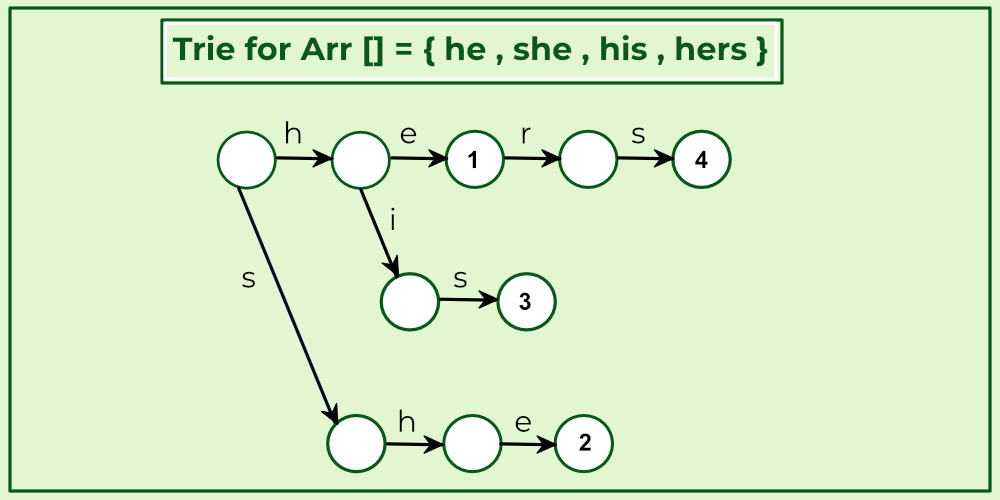Build a Trie (or Keyword Tree) of all words.

• This part fills entries in goto g[][] and output o[].
• Next, we extend Trie into an automaton to support linear time matching.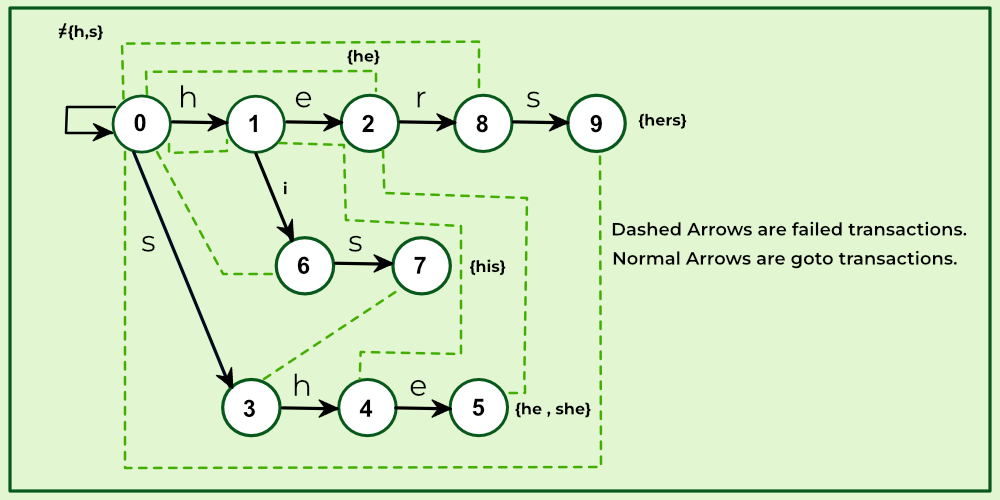Fills entries in goto g[][] and output o[]

• This part fills entries in failure f[] and output o[].

Go to:  We build Trie. And for all characters which don’t have an edge at the root, we add an edge back to root.
Failure:  For a state s, we find the longest proper suffix which is a proper prefix of some pattern. This is done using Breadth First Traversal of Trie.
Output: For a state s, indexes of all words ending at s are stored. These indexes are stored as bitwise map (by doing bitwise OR of values). This is also computing using Breadth First Traversal with Failure.

Below is the implementation of the Aho-Corasick Algorithm:

## C++

 `// C++ program for implementation of ` `// Aho Corasick algorithm for String ` `// matching ` `#include ` `using` `namespace` `std; ` ` `  `// Max number of states in the matching ` `// machine. Should be equal to the sum ` `// of the length of all keywords. ` ` `  `#define MAXS 500 ` ` `  `// Maximum number of characters ` `// in input alphabet ` ` `  `#define MAXC 26 ` ` `  `// OUTPUT FUNCTION IS IMPLEMENTED USING out[] ` `// Bit i in this mask is one if the word with ` `// index i appears when the machine enters ` `// this state. ` `int` `out[MAXS]; ` ` `  `// FAILURE FUNCTION IS IMPLEMENTED USING f[] ` `int` `f[MAXS]; ` ` `  `// GOTO FUNCTION (OR TRIE) IS ` `// IMPLEMENTED USING g[][] ` `int` `g[MAXS][MAXC]; ` ` `  `// Builds the String matching machine. ` `// arr - array of words. The index of each keyword is ` `// important: ` `//"out[state] & (1 << i)" is > 0 if we just found ` `// word[i] in the text. ` `// Returns the number of states that the built machine ` `// has. States are numbered 0 up to the return value - ` `// 1, inclusive. ` ` `  `int` `buildMatchingMachine(string arr[], ``int` `k) ` `{ ` `    ``// Initialize all values in output function as 0. ` `    ``memset``(out, 0, ``sizeof` `out); ` ` `  `    ``// Initialize all values in goto function as -1. ` `    ``memset``(g, -1, ``sizeof` `g); ` ` `  `    ``// Initially, we just have the 0 state ` `    ``int` `states = 1; ` ` `  `    ``// Convalues for goto function, i.e., fill g[][] ` `    ``// This is same as building a Trie for arr[] ` `    ``for` `(``int` `i = 0; i < k; i++) { ` `        ``string word = arr[i]; ` `        ``int` `currentState = 0; ` ` `  `        ``// Insert all characters of current ` `        ``// word in arr[] ` `        ``for` `(``int` `j = 0; j < word.length(); j++) { ` `            ``int` `ch = word[j] - ``'a'``; ` ` `  `            ``// Allocate a new node (create a new state) ` `            ``// if a node for ch doesn't exist. ` `            ``if` `(g[currentState][ch] == -1) ` `                ``g[currentState][ch] = states++; ` ` `  `            ``currentState = g[currentState][ch]; ` `        ``} ` ` `  `        ``// Add current word in output function ` `        ``out[currentState] |= (1 << i); ` `    ``} ` ` `  `    ``// For all characters which don't have ` `    ``// an edge from root (or state 0) in Trie, ` `    ``// add a goto edge to state 0 itself ` `    ``for` `(``int` `ch = 0; ch < MAXC; ch++) ` `        ``if` `(g[ch] == -1) ` `            ``g[ch] = 0; ` ` `  `    ``// Now, let's build the failure function ` `    ``// Initialize values in fail function ` `    ``memset``(f, -1, ``sizeof` `f); ` ` `  `    ``// Failure function is computed in ` `    ``// breadth first order ` `    ``// using a queue ` `    ``queue<``int``> q; ` ` `  `    ``// Iterate over every possible input ` `    ``for` `(``int` `ch = 0; ch < MAXC; ch++) { ` ` `  `        ``// All nodes of depth 1 have failure ` `        ``// function value as 0. For example, ` `        ``// in above diagram we move to 0 ` `        ``// from states 1 and 3. ` `        ``if` `(g[ch] != 0) { ` `            ``f[g[ch]] = 0; ` `            ``q.push(g[ch]); ` `        ``} ` `    ``} ` ` `  `    ``// Now queue has states 1 and 3 ` `    ``while` `(!q.empty()) { ` ` `  `        ``// Remove the front state from queue ` `        ``int` `state = q.front(); ` `        ``q.pop(); ` ` `  `        ``// For the removed state, find failure ` `        ``// function for all those characters ` `        ``// for which goto function is ` `        ``// not defined. ` `        ``for` `(``int` `ch = 0; ch < MAXC; ch++) { ` ` `  `            ``// If goto function is defined for ` `            ``// character 'ch' and 'state' ` `            ``if` `(g[state][ch] != -1) { ` ` `  `                ``// Find failure state of removed state ` `                ``int` `failure = f[state]; ` ` `  `                ``// Find the deepest node labeled by ` `                ``// proper suffix of String from root to ` `                ``// current state. ` `                ``while` `(g[failure][ch] == -1) ` `                    ``failure = f[failure]; ` `                ``failure = g[failure][ch]; ` `                ``f[g[state][ch]] = failure; ` ` `  `                ``// Merge output values ` `                ``out[g[state][ch]] |= out[failure]; ` ` `  `                ``// Insert the next level node ` `                ``// (of Trie) in Queue ` `                ``q.push(g[state][ch]); ` `            ``} ` `        ``} ` `    ``} ` `    ``return` `states; ` `} ` ` `  `// Returns the next state the machine will transition to ` `// using goto and failure functions. currentState - The ` `// current state of the machine. Must be between ` `// 0 and the number of states - 1, ` `// inclusive. ` `// nextInput - The next character that enters into the ` `// machine. ` ` `  `// This function finds all occurrences of ` `// all array words in text. ` `void` `searchWords(string arr[], ``int` `k, string text) ` `{ ` ` `  `    ``// Preprocess patterns. ` `    ``// Build machine with goto, failure ` `    ``// and output functions ` `    ``buildMatchingMachine(arr, k); ` ` `  `    ``// Initialize current state ` `    ``int` `currentState = 0; ` ` `  `    ``// Traverse the text through the ` `    ``// built machine to find all ` `    ``// occurrences of words in arr[] ` `    ``for` `(``int` `i = 0; i < text.length(); i++) { ` `        ``int` `ch = text[i] - ``'a'``; ` ` `  `        ``// If goto is not defined, use ` `        ``// failure function ` `        ``while` `(g[currentState][ch] == -1) ` `            ``currentState = f[currentState]; ` `        ``currentState = g[currentState][ch]; ` ` `  `        ``// If match not found, move to next state ` `        ``if` `(out[currentState] == 0) ` `            ``continue``; ` ` `  `        ``// Match found, print all matching ` `        ``// words of arr[] ` `        ``// using output function. ` `        ``for` `(``int` `j = 0; j < k; j++) { ` `            ``if` `(out[currentState] & (1 << j)) ` `                ``cout << ``"Word "` `<< arr[j] ` `                     ``<< ``" appears from "` `                     ``<< i - arr[j].length() + 1 << ``" to "` `                     ``<< i << endl; ` `        ``} ` `    ``} ` `} ` `// Driver code ` ` `  `int` `main() ` `{ ` `    ``string arr[] = { ``"he"``, ``"she"``, ``"hers"``, ``"his"` `}; ` `    ``int` `k = ``sizeof``(arr) / ``sizeof``(arr); ` `    ``string text = ``"ahishers"``; ` `    ``searchWords(arr, k, text); ` `    ``return` `0; ` `}`

## Java

 `// Java program for implementation of ` `// Aho Corasick algorithm for String ` `// matching ` `import` `java.util.*; ` ` `  `class` `GFG { ` ` `  `    ``// Max number of states in the matching ` `    ``// machine. Should be equal to the sum ` `    ``// of the length of all keywords. ` `    ``static` `int` `MAXS = ``500``; ` ` `  `    ``// Maximum number of characters ` `    ``// in input alphabet ` `    ``static` `int` `MAXC = ``26``; ` ` `  `    ``// OUTPUT FUNCTION IS IMPLEMENTED USING out[] ` `    ``// Bit i in this mask is one if the word with ` `    ``// index i appears when the machine enters ` `    ``// this state. ` `    ``static` `int``[] out = ``new` `int``[MAXS]; ` ` `  `    ``// FAILURE FUNCTION IS IMPLEMENTED USING f[] ` `    ``static` `int``[] f = ``new` `int``[MAXS]; ` ` `  `    ``// GOTO FUNCTION (OR TRIE) IS ` `    ``// IMPLEMENTED USING g[][] ` `    ``static` `int``[][] g = ``new` `int``[MAXS][MAXC]; ` ` `  `    ``// Builds the String matching machine. ` `    ``// arr - array of words. The index of each keyword is ` `    ``// important: ` `    ``//         "out[state] & (1 << i)" is > 0 if we just ` `    ``//         found ` `    ``// word[i]          in the text. ` `    ``// Returns the number of states that the built machine ` `    ``// has. States are numbered 0 up to the return value - ` `    ``// 1, inclusive. ` `    ``static` `int` `buildMatchingMachine(String arr[], ``int` `k) ` `    ``{ ` ` `  `        ``// Initialize all values in output function as 0. ` `        ``Arrays.fill(out, ``0``); ` ` `  `        ``// Initialize all values in goto function as -1. ` `        ``for` `(``int` `i = ``0``; i < MAXS; i++) ` `            ``Arrays.fill(g[i], -``1``); ` ` `  `        ``// Initially, we just have the 0 state ` `        ``int` `states = ``1``; ` ` `  `        ``// Convalues for goto function, i.e., fill g[][] ` `        ``// This is same as building a Trie for arr[] ` `        ``for` `(``int` `i = ``0``; i < k; ++i) { ` `            ``String word = arr[i]; ` `            ``int` `currentState = ``0``; ` ` `  `            ``// Insert all characters of current ` `            ``// word in arr[] ` `            ``for` `(``int` `j = ``0``; j < word.length(); ++j) { ` `                ``int` `ch = word.charAt(j) - ``'a'``; ` ` `  `                ``// Allocate a new node (create a new state) ` `                ``// if a node for ch doesn't exist. ` `                ``if` `(g[currentState][ch] == -``1``) ` `                    ``g[currentState][ch] = states++; ` ` `  `                ``currentState = g[currentState][ch]; ` `            ``} ` ` `  `            ``// Add current word in output function ` `            ``out[currentState] |= (``1` `<< i); ` `        ``} ` ` `  `        ``// For all characters which don't have ` `        ``// an edge from root (or state 0) in Trie, ` `        ``// add a goto edge to state 0 itself ` `        ``for` `(``int` `ch = ``0``; ch < MAXC; ++ch) ` `            ``if` `(g[``0``][ch] == -``1``) ` `                ``g[``0``][ch] = ``0``; ` ` `  `        ``// Now, let's build the failure function ` `        ``// Initialize values in fail function ` `        ``Arrays.fill(f, -``1``); ` ` `  `        ``// Failure function is computed in ` `        ``// breadth first order ` `        ``// using a queue ` `        ``Queue q = ``new` `LinkedList<>(); ` ` `  `        ``// Iterate over every possible input ` `        ``for` `(``int` `ch = ``0``; ch < MAXC; ++ch) { ` ` `  `            ``// All nodes of depth 1 have failure ` `            ``// function value as 0. For example, ` `            ``// in above diagram we move to 0 ` `            ``// from states 1 and 3. ` `            ``if` `(g[``0``][ch] != ``0``) { ` `                ``f[g[``0``][ch]] = ``0``; ` `                ``q.add(g[``0``][ch]); ` `            ``} ` `        ``} ` ` `  `        ``// Now queue has states 1 and 3 ` `        ``while` `(!q.isEmpty()) { ` ` `  `            ``// Remove the front state from queue ` `            ``int` `state = q.peek(); ` `            ``q.remove(); ` ` `  `            ``// For the removed state, find failure ` `            ``// function for all those characters ` `            ``// for which goto function is ` `            ``// not defined. ` `            ``for` `(``int` `ch = ``0``; ch < MAXC; ++ch) { ` ` `  `                ``// If goto function is defined for ` `                ``// character 'ch' and 'state' ` `                ``if` `(g[state][ch] != -``1``) { ` ` `  `                    ``// Find failure state of removed state ` `                    ``int` `failure = f[state]; ` ` `  `                    ``// Find the deepest node labeled by ` `                    ``// proper suffix of String from root to ` `                    ``// current state. ` `                    ``while` `(g[failure][ch] == -``1``) ` `                        ``failure = f[failure]; ` ` `  `                    ``failure = g[failure][ch]; ` `                    ``f[g[state][ch]] = failure; ` ` `  `                    ``// Merge output values ` `                    ``out[g[state][ch]] |= out[failure]; ` ` `  `                    ``// Insert the next level node ` `                    ``// (of Trie) in Queue ` `                    ``q.add(g[state][ch]); ` `                ``} ` `            ``} ` `        ``} ` `        ``return` `states; ` `    ``} ` ` `  `    ``// Returns the next state the machine will transition to ` `    ``// using goto and failure functions. currentState - The ` `    ``// current state of the machine. Must be between ` `    ``// 0 and the number of states - 1, ` `    ``// inclusive. ` `    ``// nextInput - The next character that enters into the ` `    ``// machine. ` `    ``static` `int` `findNextState(``int` `currentState, ` `                             ``char` `nextInput) ` `    ``{ ` `        ``int` `answer = currentState; ` `        ``int` `ch = nextInput - ``'a'``; ` ` `  `        ``// If goto is not defined, use ` `        ``// failure function ` `        ``while` `(g[answer][ch] == -``1``) ` `            ``answer = f[answer]; ` ` `  `        ``return` `g[answer][ch]; ` `    ``} ` ` `  `    ``// This function finds all occurrences of ` `    ``// all array words in text. ` `    ``static` `void` `searchWords(String arr[], ``int` `k, ` `                            ``String text) ` `    ``{ ` ` `  `        ``// Preprocess patterns. ` `        ``// Build machine with goto, failure ` `        ``// and output functions ` `        ``buildMatchingMachine(arr, k); ` ` `  `        ``// Initialize current state ` `        ``int` `currentState = ``0``; ` ` `  `        ``// Traverse the text through the ` `        ``// built machine to find all ` `        ``// occurrences of words in arr[] ` `        ``for` `(``int` `i = ``0``; i < text.length(); ++i) { ` `            ``currentState = findNextState(currentState, ` `                                         ``text.charAt(i)); ` ` `  `            ``// If match not found, move to next state ` `            ``if` `(out[currentState] == ``0``) ` `                ``continue``; ` ` `  `            ``// Match found, print all matching ` `            ``// words of arr[] ` `            ``// using output function. ` `            ``for` `(``int` `j = ``0``; j < k; ++j) { ` `                ``if` `((out[currentState] & (``1` `<< j)) > ``0``) { ` `                    ``System.out.print( ` `                        ``"Word "` `+ arr[j] + ``" appears from "` `                        ``+ (i - arr[j].length() + ``1``) + ``" to "` `                        ``+ i + ``"\n"``); ` `                ``} ` `            ``} ` `        ``} ` `    ``} ` ` `  `    ``// Driver code ` `    ``public` `static` `void` `main(String[] args) ` `    ``{ ` `        ``String arr[] = { ``"he"``, ``"she"``, ``"hers"``, ``"his"` `}; ` `        ``String text = ``"ahishers"``; ` `        ``int` `k = arr.length; ` ` `  `        ``searchWords(arr, k, text); ` `    ``} ` `} ` `// This code is wriiten by Sundaram.`

## Python

 `# Python program for implementation of ` `# Aho-Corasick algorithm for string matching ` ` `  `# defaultdict is used only for storing the final output ` `# We will return a dictionary where key is the matched word ` `# and value is the list of indexes of matched word ` `from` `collections ``import` `defaultdict ` ` `  `# For simplicity, Arrays and Queues have been implemented using lists. ` `# If you want to improve performance try using them instead ` ` `  ` `  `class` `AhoCorasick: ` `    ``def` `__init__(``self``, words): ` ` `  `        ``# Max number of states in the matching machine. ` `        ``# Should be equal to the sum of the length of all keywords. ` `        ``self``.max_states ``=` `sum``([``len``(word) ``for` `word ``in` `words]) ` ` `  `        ``# Maximum number of characters. ` `        ``# Currently supports only alphabets [a, z] ` `        ``self``.max_characters ``=` `26` ` `  `        ``# OUTPUT FUNCTION IS IMPLEMENTED USING out [] ` `        ``# Bit i in this mask is 1 if the word with ` `        ``# index i appears when the machine enters this state. ` `        ``# Lets say, a state outputs two words "he" and "she" and ` `        ``# in our provided words list, he has index 0 and she has index 3 ` `        ``# so value of out[state] for this state will be 1001 ` `        ``# It has been initialized to all 0. ` `        ``# We have taken one extra state for the root. ` `        ``self``.out ``=` `[``0``]``*``(``self``.max_states ``+` `1``) ` ` `  `        ``# FAILURE FUNCTION IS IMPLEMENTED USING fail [] ` `        ``# There is one value for each state + 1 for the root ` `        ``# It has been initialized to all -1 ` `        ``# This will contain the fail state value for each state ` `        ``self``.fail ``=` `[``-``1``]``*``(``self``.max_states ``+` `1``) ` ` `  `        ``# GOTO FUNCTION (OR TRIE) IS IMPLEMENTED USING goto [[]] ` `        ``# Number of rows = max_states + 1 ` `        ``# Number of columns = max_characters i.e 26 in our case ` `        ``# It has been initialized to all -1. ` `        ``self``.goto ``=` `[ ` `            ``[``-``1``]``*``self``.max_characters ``for` `_ ``in` `range``(``self``.max_states ``+` `1``)] ` ` `  `        ``# Convert all words to lowercase ` `        ``# so that our search is case insensitive ` `        ``for` `i ``in` `range``(``len``(words)): ` `            ``words[i] ``=` `words[i].lower() ` ` `  `        ``# All the words in dictionary which will be used to create Trie ` `        ``# The index of each keyword is important: ` `        ``# "out[state] & (1 << i)" is > 0 if we just found word[i] ` `        ``# in the text. ` `        ``self``.words ``=` `words ` ` `  `        ``# Once the Trie has been built, it will contain the number ` `        ``# of nodes in Trie which is total number of states required <= max_states ` `        ``self``.states_count ``=` `self``.__build_matching_machine() ` ` `  `    ``# Builds the String matching machine. ` `    ``# Returns the number of states that the built machine has. ` `    ``# States are numbered 0 up to the return value - 1, inclusive. ` ` `  `    ``def` `__build_matching_machine(``self``): ` `        ``k ``=` `len``(``self``.words) ` ` `  `        ``# Initially, we just have the 0 state ` `        ``states ``=` `1` ` `  `        ``# Convalues for goto function, i.e., fill goto ` `        ``# This is same as building a Trie for words[] ` `        ``for` `i ``in` `range``(k): ` `            ``word ``=` `self``.words[i] ` `            ``current_state ``=` `0` ` `  `            ``# Process all the characters of the current word ` `            ``for` `character ``in` `word: ` `                ``ch ``=` `ord``(character) ``-` `97`  `# Ascii value of 'a' = 97 ` ` `  `                ``# Allocate a new node (create a new state) ` `                ``# if a node for ch doesn't exist. ` `                ``if` `self``.goto[current_state][ch] ``=``=` `-``1``: ` `                    ``self``.goto[current_state][ch] ``=` `states ` `                    ``states ``+``=` `1` ` `  `                ``current_state ``=` `self``.goto[current_state][ch] ` ` `  `            ``# Add current word in output function ` `            ``self``.out[current_state] |``=` `(``1` `<< i) ` ` `  `        ``# For all characters which don't have ` `        ``# an edge from root (or state 0) in Trie, ` `        ``# add a goto edge to state 0 itself ` `        ``for` `ch ``in` `range``(``self``.max_characters): ` `            ``if` `self``.goto[``0``][ch] ``=``=` `-``1``: ` `                ``self``.goto[``0``][ch] ``=` `0` ` `  `        ``# Failure function is computed in ` `        ``# breadth first order using a queue ` `        ``queue ``=` `[] ` ` `  `        ``# Iterate over every possible input ` `        ``for` `ch ``in` `range``(``self``.max_characters): ` ` `  `            ``# All nodes of depth 1 have failure ` `            ``# function value as 0. For example, ` `            ``# in above diagram we move to 0 ` `            ``# from states 1 and 3. ` `            ``if` `self``.goto[``0``][ch] !``=` `0``: ` `                ``self``.fail[``self``.goto[``0``][ch]] ``=` `0` `                ``queue.append(``self``.goto[``0``][ch]) ` ` `  `        ``# Now queue has states 1 and 3 ` `        ``while` `queue: ` ` `  `            ``# Remove the front state from queue ` `            ``state ``=` `queue.pop(``0``) ` ` `  `            ``# For the removed state, find failure ` `            ``# function for all those characters ` `            ``# for which goto function is not defined. ` `            ``for` `ch ``in` `range``(``self``.max_characters): ` ` `  `                ``# If goto function is defined for ` `                ``# character 'ch' and 'state' ` `                ``if` `self``.goto[state][ch] !``=` `-``1``: ` ` `  `                    ``# Find failure state of removed state ` `                    ``failure ``=` `self``.fail[state] ` ` `  `                    ``# Find the deepest node labeled by proper ` `                    ``# suffix of String from root to current state. ` `                    ``while` `self``.goto[failure][ch] ``=``=` `-``1``: ` `                        ``failure ``=` `self``.fail[failure] ` ` `  `                    ``failure ``=` `self``.goto[failure][ch] ` `                    ``self``.fail[``self``.goto[state][ch]] ``=` `failure ` ` `  `                    ``# Merge output values ` `                    ``self``.out[``self``.goto[state][ch]] |``=` `self``.out[failure] ` ` `  `                    ``# Insert the next level node (of Trie) in Queue ` `                    ``queue.append(``self``.goto[state][ch]) ` ` `  `        ``return` `states ` ` `  `    ``# Returns the next state the machine will transition to using goto ` `    ``# and failure functions. ` `    ``# current_state - The current state of the machine. Must be between ` `    ``# 0 and the number of states - 1, inclusive. ` `    ``# next_input - The next character that enters into the machine. ` ` `  `    ``def` `__find_next_state(``self``, current_state, next_input): ` `        ``answer ``=` `current_state ` `        ``ch ``=` `ord``(next_input) ``-` `97`  `# Ascii value of 'a' is 97 ` ` `  `        ``# If goto is not defined, use ` `        ``# failure function ` `        ``while` `self``.goto[answer][ch] ``=``=` `-``1``: ` `            ``answer ``=` `self``.fail[answer] ` ` `  `        ``return` `self``.goto[answer][ch] ` ` `  `    ``# This function finds all occurrences of all words in text. ` ` `  `    ``def` `search_words(``self``, text): ` `        ``# Convert the text to lowercase to make search case insensitive ` `        ``text ``=` `text.lower() ` ` `  `        ``# Initialize current_state to 0 ` `        ``current_state ``=` `0` ` `  `        ``# A dictionary to store the result. ` `        ``# Key here is the found word ` `        ``# Value is a list of all occurrences start index ` `        ``result ``=` `defaultdict(``list``) ` ` `  `        ``# Traverse the text through the built machine ` `        ``# to find all occurrences of words ` `        ``for` `i ``in` `range``(``len``(text)): ` `            ``current_state ``=` `self``.__find_next_state(current_state, text[i]) ` ` `  `            ``# If match not found, move to next state ` `            ``if` `self``.out[current_state] ``=``=` `0``: ` `                ``continue` ` `  `            ``# Match found, store the word in result dictionary ` `            ``for` `j ``in` `range``(``len``(``self``.words)): ` `                ``if` `(``self``.out[current_state] & (``1` `<< j)) > ``0``: ` `                    ``word ``=` `self``.words[j] ` ` `  `                    ``# Start index of word is (i-len(word)+1) ` `                    ``result[word].append(i``-``len``(word)``+``1``) ` ` `  `        ``# Return the final result dictionary ` `        ``return` `result ` ` `  ` `  `# Driver code ` `if` `__name__ ``=``=` `"__main__"``: ` `    ``words ``=` `[``"he"``, ``"she"``, ``"hers"``, ``"his"``] ` `    ``text ``=` `"ahishers"` ` `  `    ``# Create an Object to initialize the Trie ` `    ``aho_chorasick ``=` `AhoCorasick(words) ` ` `  `    ``# Get the result ` `    ``result ``=` `aho_chorasick.search_words(text) ` ` `  `    ``# Print the result ` `    ``for` `word ``in` `result: ` `        ``for` `i ``in` `result[word]: ` `            ``print``(``"Word"``, word, ``"appears from"``, i, ``"to"``, i ``+` `len``(word)``-``1``) `

## Javascript

 `const MAXS = 500; ` `const MAXC = 26; ` ` `  `let out = ``new` `Array(MAXS).fill(0); ` `let f = ``new` `Array(MAXS).fill(-1); ` `let g = Array.from(Array(MAXS), () => ``new` `Array(MAXC).fill(-1)); ` ` `  `function` `buildMatchingMachine(arr, k) { ` `  ``out.fill(0); ` `  ``g.forEach(row => row.fill(-1)); ` `  ``let states = 1; ` `  ``for` `(let i = 0; i < k; i++) { ` `    ``const word = arr[i]; ` `    ``let currentState = 0; ` `    ``for` `(let j = 0; j < word.length; j++) { ` `      ``const ch = word.charCodeAt(j) - ``'a'``.charCodeAt(0); ` `      ``if` `(g[currentState][ch] === -1) g[currentState][ch] = states++; ` `      ``currentState = g[currentState][ch]; ` `    ``} ` `    ``out[currentState] |= 1 << i; ` `  ``} ` `  ``for` `(let ch = 0; ch < MAXC; ch++) { ` `    ``if` `(g[ch] === -1) g[ch] = 0; ` `  ``} ` `  ``f.fill(-1); ` `  ``const q = []; ` `  ``for` `(let ch = 0; ch < MAXC; ch++) { ` `    ``if` `(g[ch] !== 0) { ` `      ``f[g[ch]] = 0; ` `      ``q.push(g[ch]); ` `    ``} ` `  ``} ` `  ``while` `(q.length) { ` `    ``const state = q.shift(); ` `    ``for` `(let ch = 0; ch < MAXC; ch++) { ` `      ``if` `(g[state][ch] !== -1) { ` `        ``let failure = f[state]; ` `        ``while` `(g[failure][ch] === -1) failure = f[failure]; ` `        ``failure = g[failure][ch]; ` `        ``f[g[state][ch]] = failure; ` `        ``out[g[state][ch]] |= out[failure]; ` `        ``q.push(g[state][ch]); ` `      ``} ` `    ``} ` `  ``} ` `  ``return` `states; ` `} ` ` `  `function` `searchWords(arr, k, text) { ` `  ``buildMatchingMachine(arr, k); ` `  ``let currentState = 0; ` `  ``for` `(let i = 0; i < text.length; i++) { ` `    ``const ch = text.charCodeAt(i) - ``'a'``.charCodeAt(0); ` `    ``while` `(g[currentState][ch] === -1) currentState = f[currentState]; ` `    ``currentState = g[currentState][ch]; ` `    ``if` `(out[currentState] === 0) ``continue``; ` `    ``for` `(let j = 0; j < k; j++) { ` `      ``if` `(out[currentState] & (1 << j)) { ` `        ``console.log(`Word \${arr[j]} appears from \${i - arr[j].length + 1} to \${i}`); ` `      ``} ` `    ``} ` `  ``} ` `} ` ` `  `// Driver code ` `const arr = [``"he"``, ``"she"``, ``"hers"``, ``"his"``]; ` `const k = arr.length; ` `const text = ``"ahishers"``; ` `searchWords(arr, k, text); `

Output

```Word his appears from 1 to 3
Word he appears from 4 to 5
Word she appears from 3 to 5
Word hers appears from 4 to 7```

Time Complexity: O(n + l + z), where ‘n’ is the length of the text, ‘l’ is the length of keywords, and ‘z’ is the number of matches.
Auxiliary Space: O(l * q), where ‘q’ is the length of the alphabet since that is the maximum number of children a node can have.

My Personal Notes arrow_drop_up